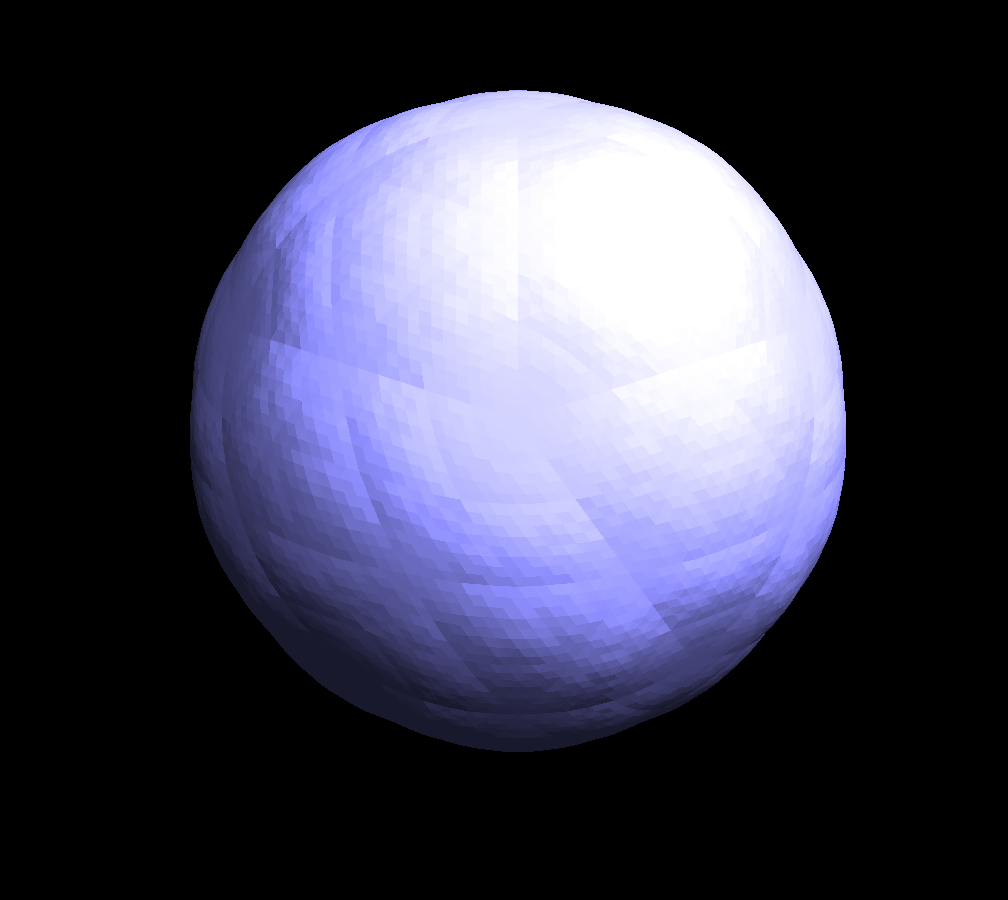# Project 2: Mesh Editor

## Shivam Parikh, CS184-Spring 2019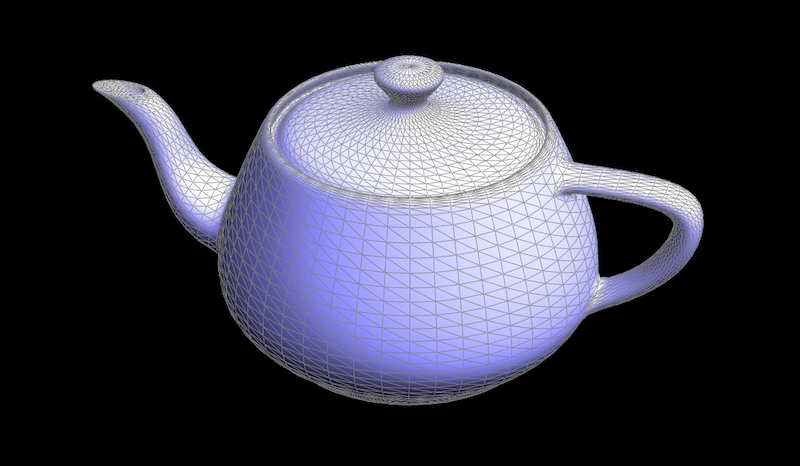## Overview

The main goal of this project was to implement a mesh editor to manipulate half-edge meshes and implement loop subdivisions to improve the resolution of the mesh. As part of the project, we also implemented de Casteljau's algorithm to display/render Bezier curves and surfaces. The final product was an editor/viewer that allowed us to load meshes and curves, flip edges, split edges, and subdivide the mesh.

## Section I: Bezier Curves and Surfaces

### Part 1: Bezier curves with 1D de Casteljau subdivision

de Casteljau's algorithm is a recursive technique to evaluate the polynomials we use to represent Bezier curves. An n degree Bezier curve is defined by (n+1) control points, which we recursively evaluate in levels to narrow from (n+1) points to a single point based on a parameter t between [0,1].
The linear interpolation step is conducted as shown by the math below: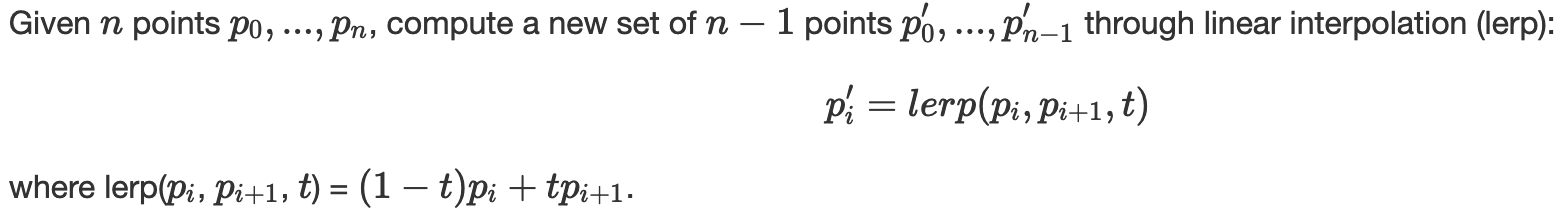We repeat these steps of linear interpolation until we narrow down to a single point for each parameter in the continuous range of t. The steps of evaluation are demonstrated by the screenshots of our editor below. In white are the original control points, and in blue are the next evaluated level of recursive points. The red point is the final point for the given parameter t.

#### The points used for the screenshots.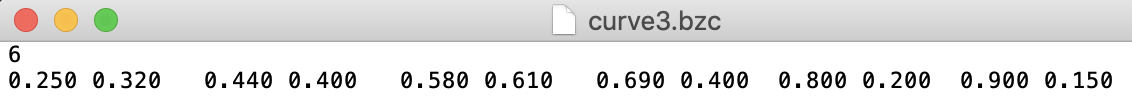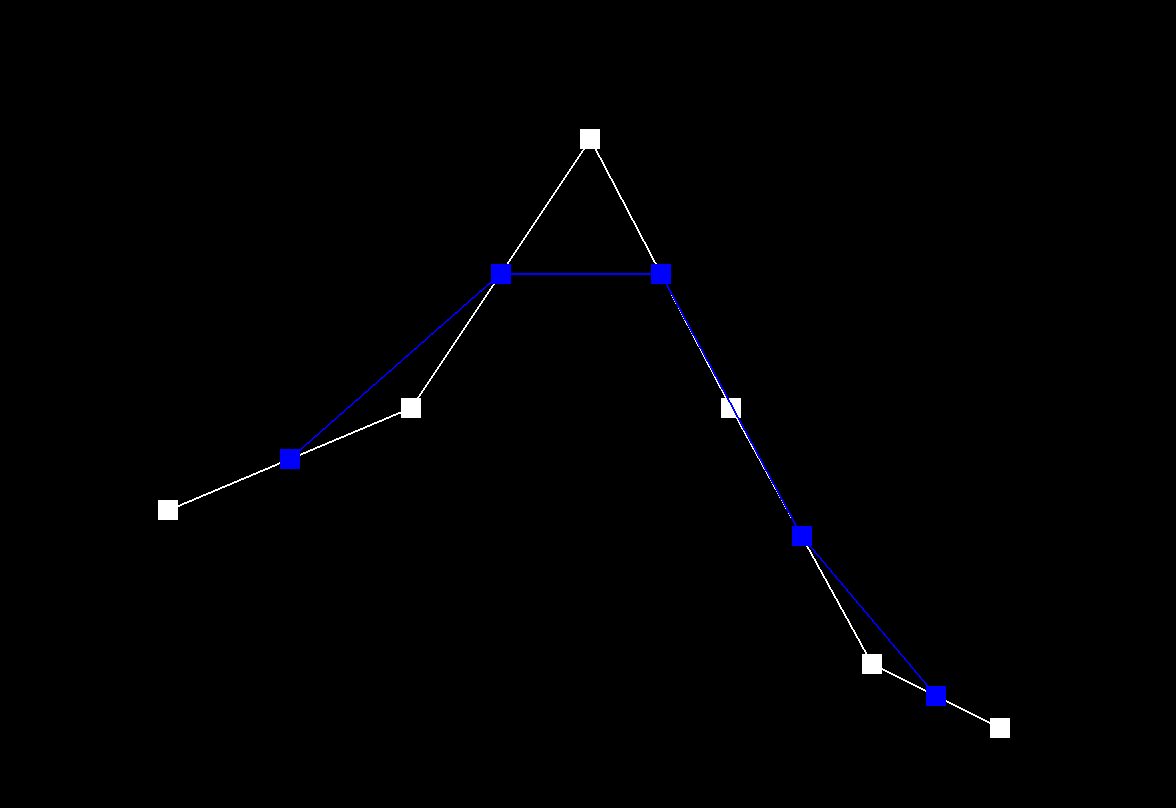The first level of evaluation.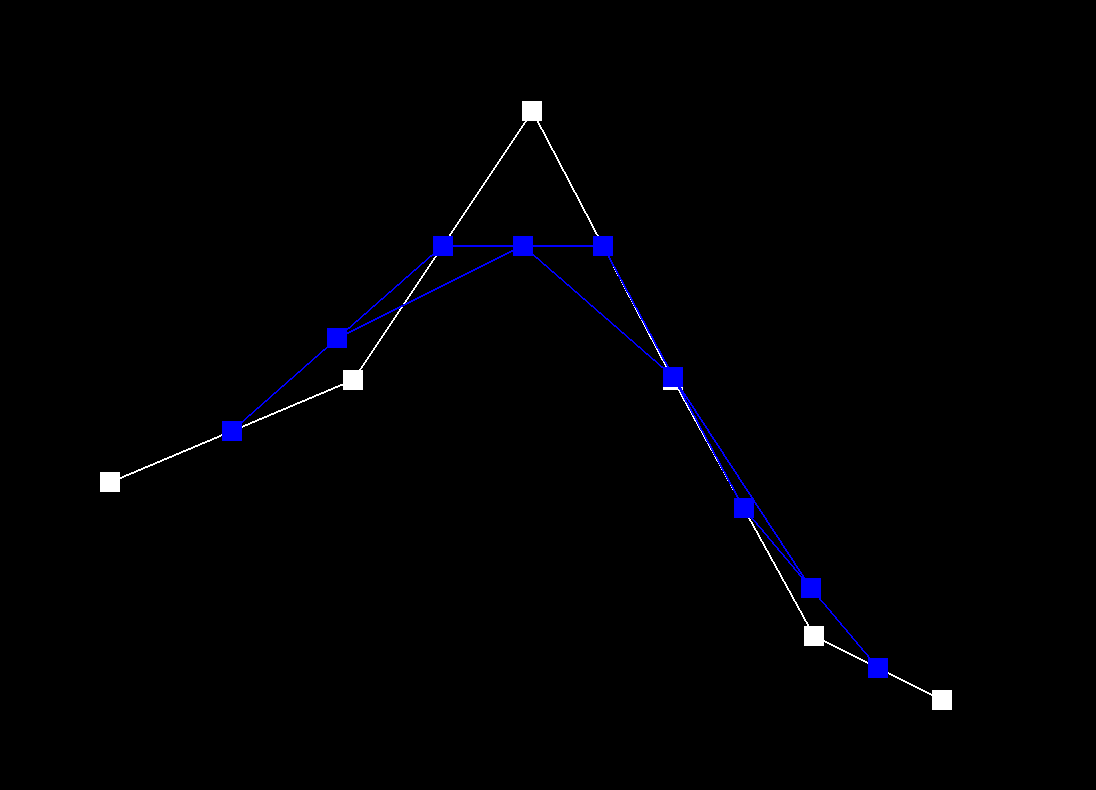The second level of evaluation.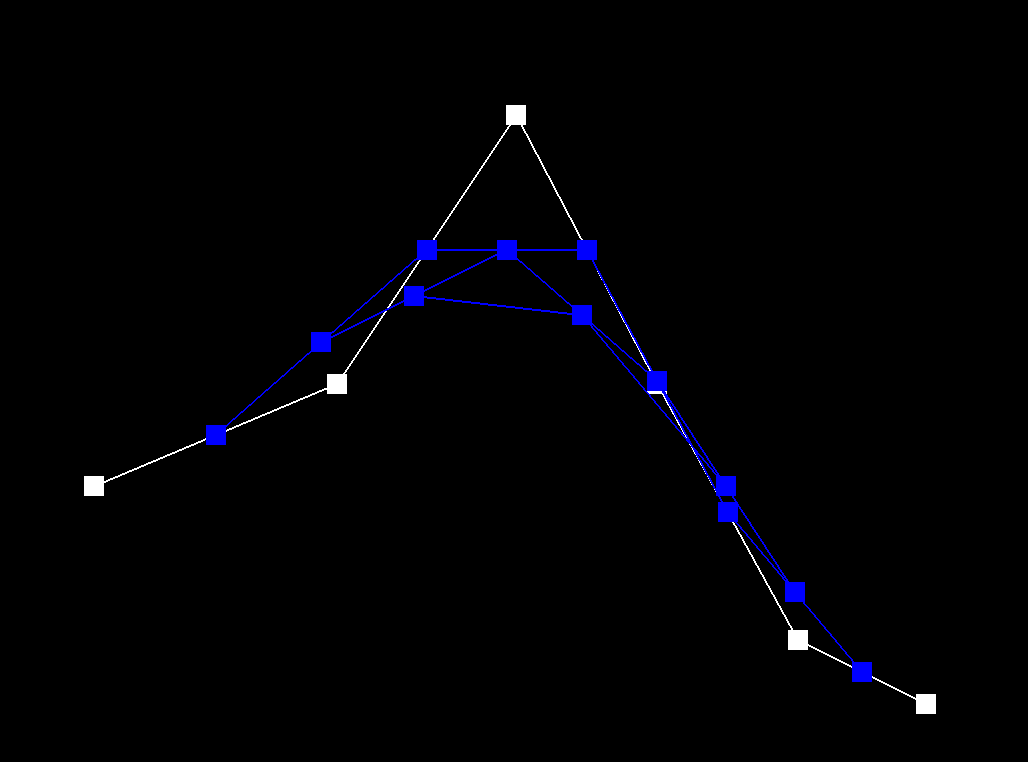The third level of evaluation.The fourth level of evaluation.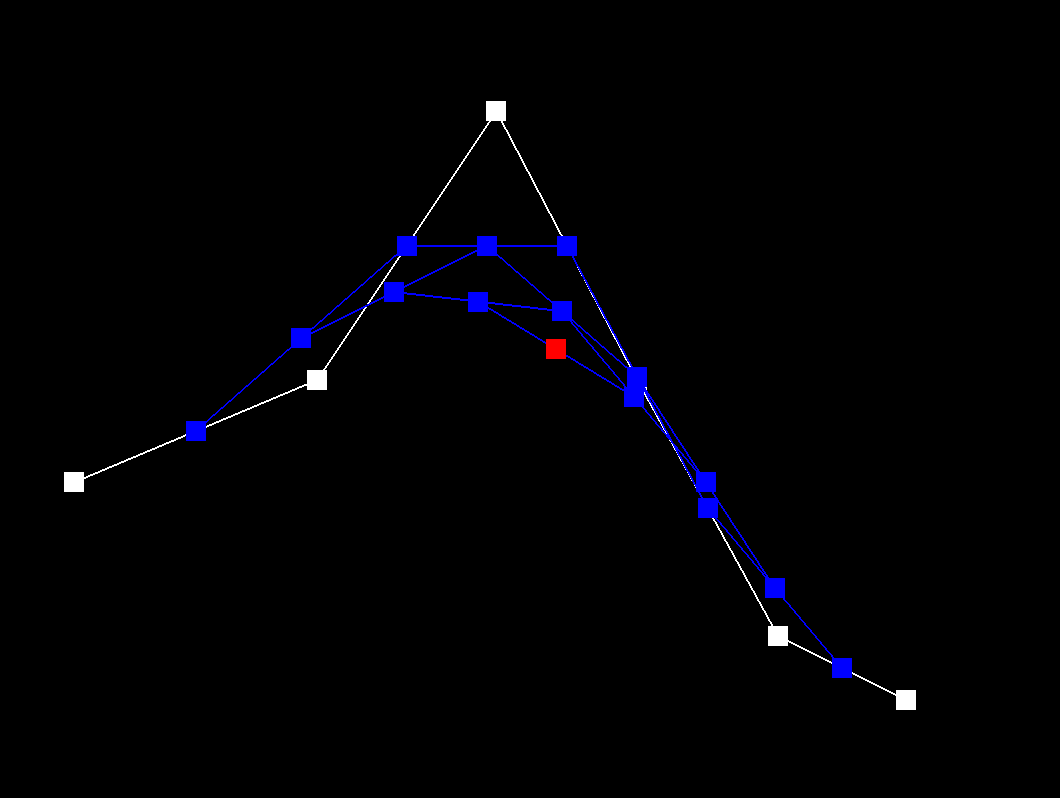The fifth level of evaluation.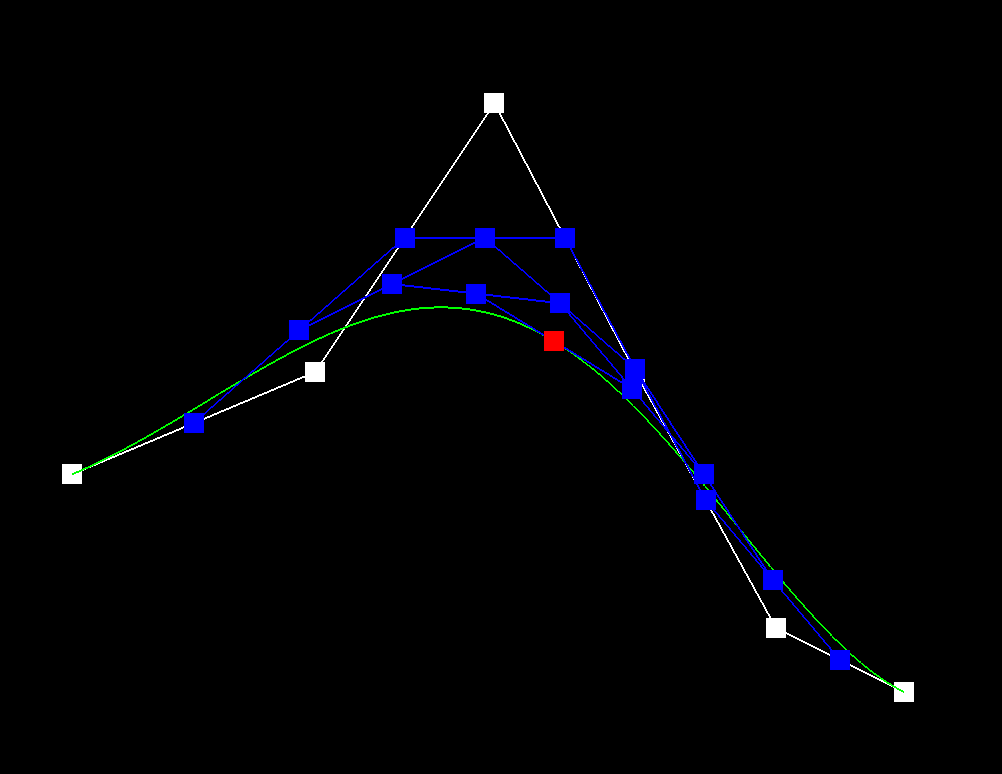The fifth level of evaluation with the actual curve evaluated at all points of t drawn.

Because our viewer is also an editor, I moved some of the points around to create a new curve, and also varied the value of the parameter t to show the evaluation at different points in time.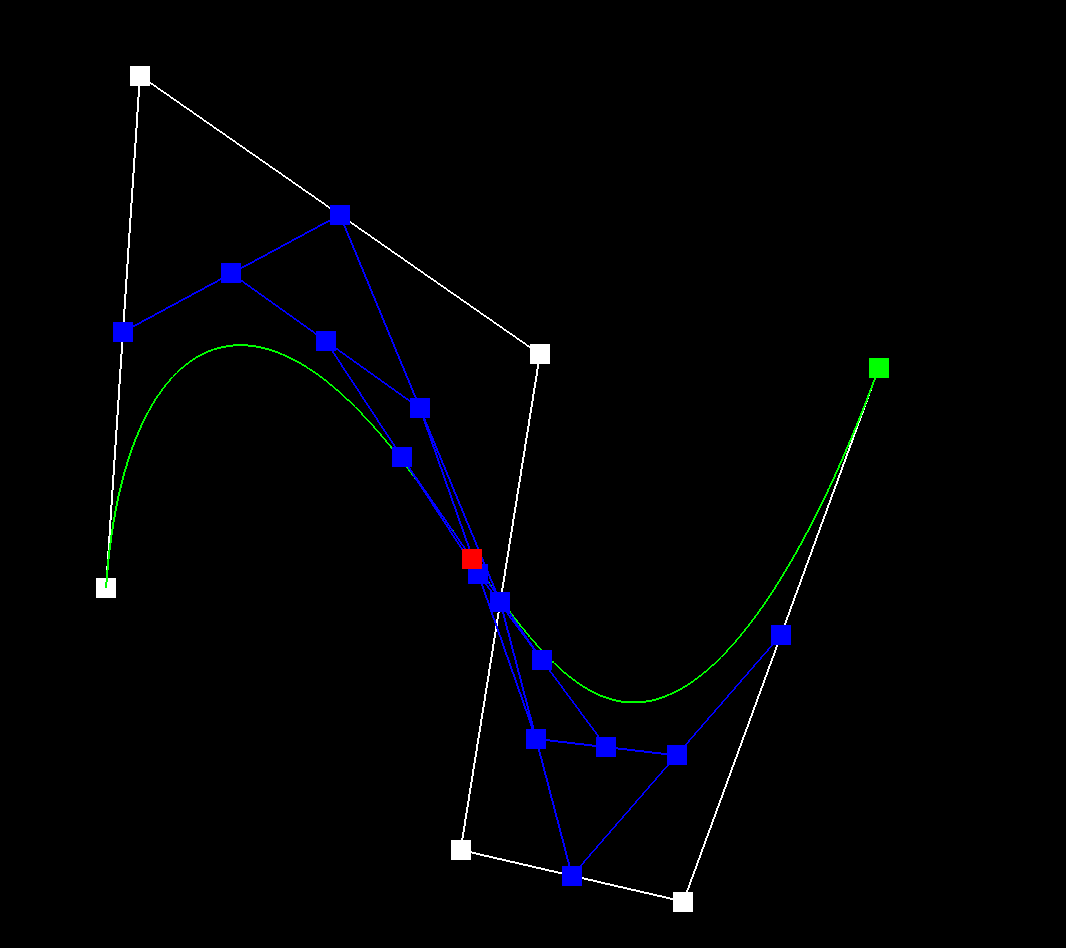The moved points.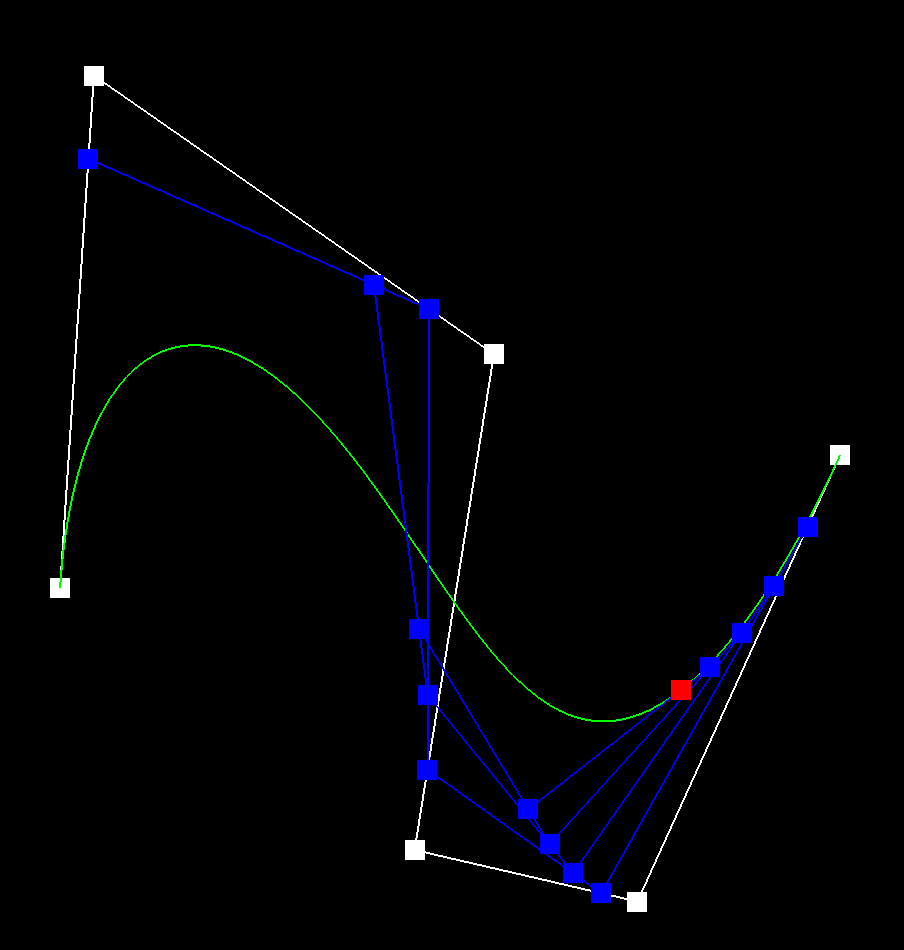The moved points at one point on the parameter t.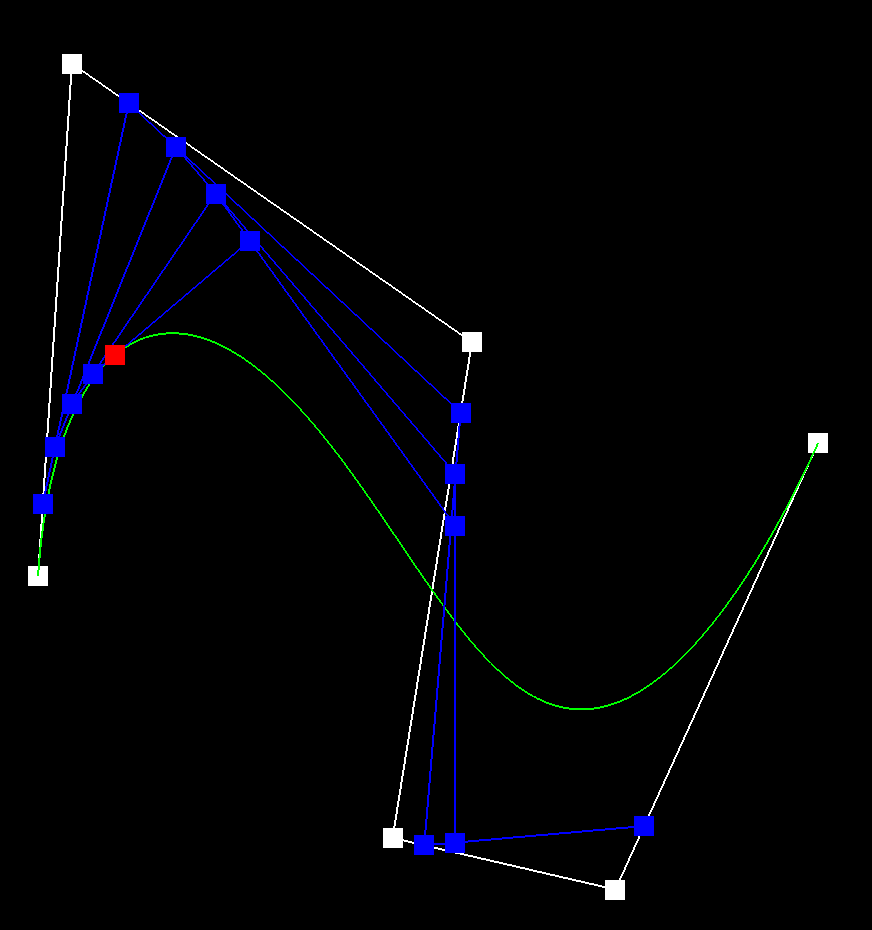The moved points at another point on the parameter t.

### Part 2: Bezier surfaces with separable 1D de Casteljau subdivision

While the last part was about two dimensional curves based on a parameter t, this part on Bezier surfaces was working with three dimensional curves to form planar surfaces. The process of evaluation was actually very similar to the first part, but just repeated. In this part, we were given a 4x4 matrix of control points defining the cubic Bezier surface. In total, there were 16 control points. We were also given two parameters, u and v. I simply replicated the two dimensional evaluation using de Casteljau that took in four control points and one parameter, and evaluated this on each row of the matrix with the parameter u. Then with the four resulting points, I evaluated using de Casteljau again, this time with the v parameter to evaluate our resultant cubic plane over the range of [0,1] very similar to the single row evaluation. The de Casteljau algorithm extends pretty simply between the 1D and 2D control points cases, as is shown by the way we evaluate over the two parameters using different rows of the matrix.
When rendering the bez/teapot.bez file, we end up with this beautiful rendering of a teapot.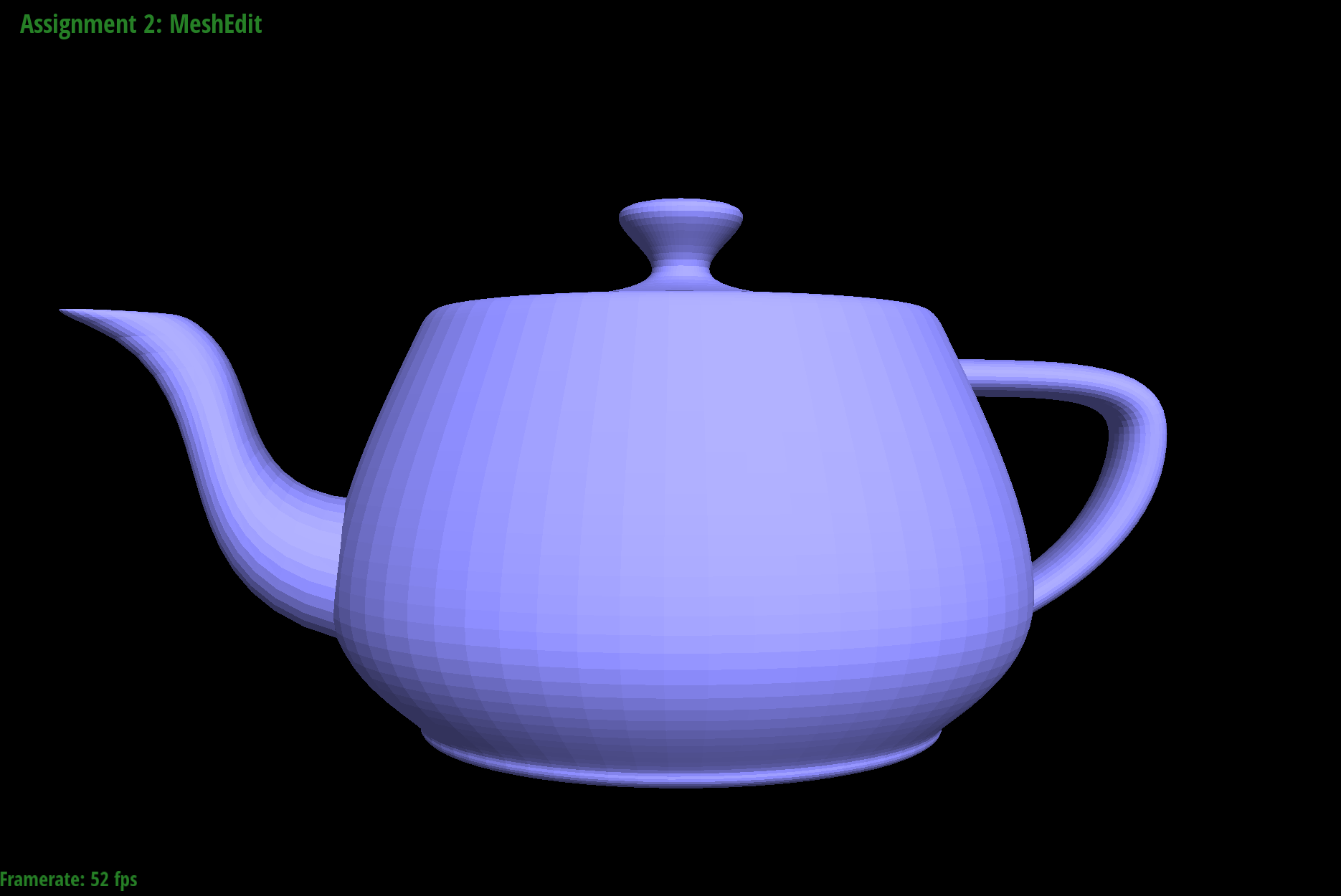And we also have another bez file, the wavy cube!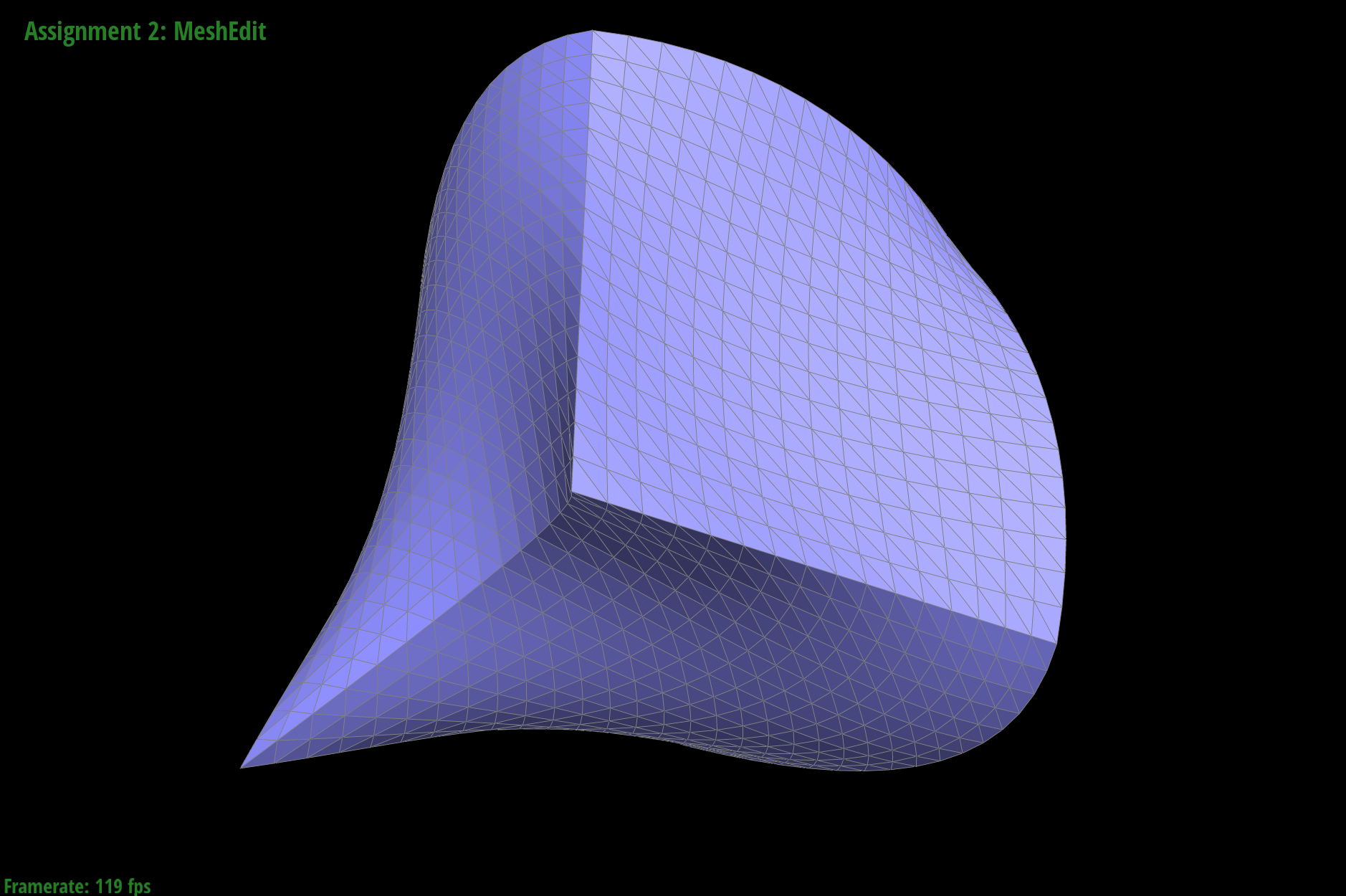## Section II: Sampling

After finishing the rendering of Bezier curves and surfaces, it was time to delve deeper into the Halfedge mesh datastructure.

### Part 3: Average normals for half-edge meshes

To start working on this part, I began by reading through the documentation for the halfedge mesh class and understanding the various class variables each attribute had. Because the Vector3D supports addition and subtraction and a unit operation, getting the area weighted average normals was not particularly difficult. After assigning the correct pointers and end condition for the while loop, I looped through all the neighboring vertices (using the halfedges and twins to find them), found the vector between the adjacent vertices and the position of the vertex we were operating from, and then summed the cross product of the two vectors (the area between the two vectors) into an accumulating Vector3D. Then normalizng the accumulating Vector3D provides us with the area-weighted average normal vector at a vertex. The results of the normal vectors are displayed below.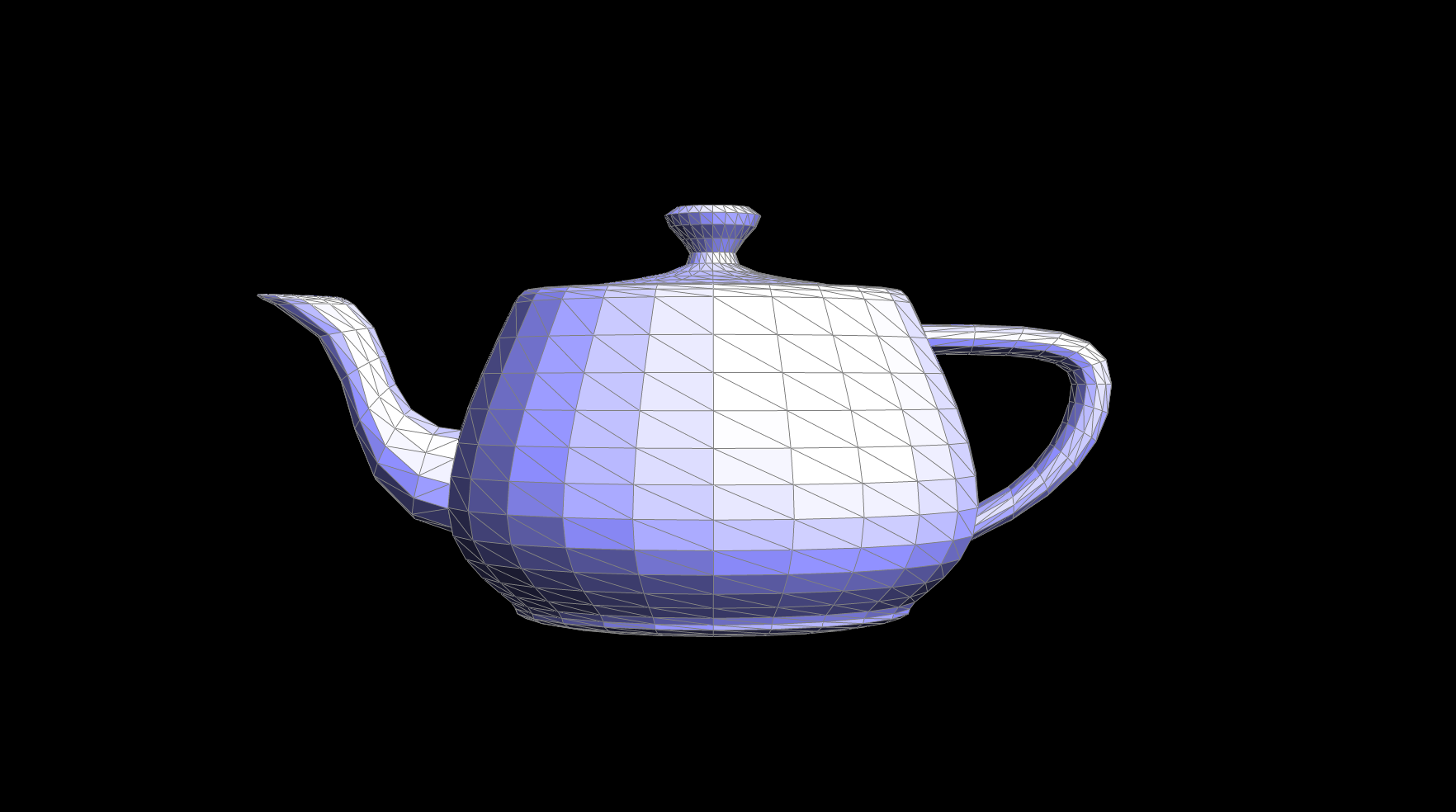With face normals.With the average vertex normal vectors.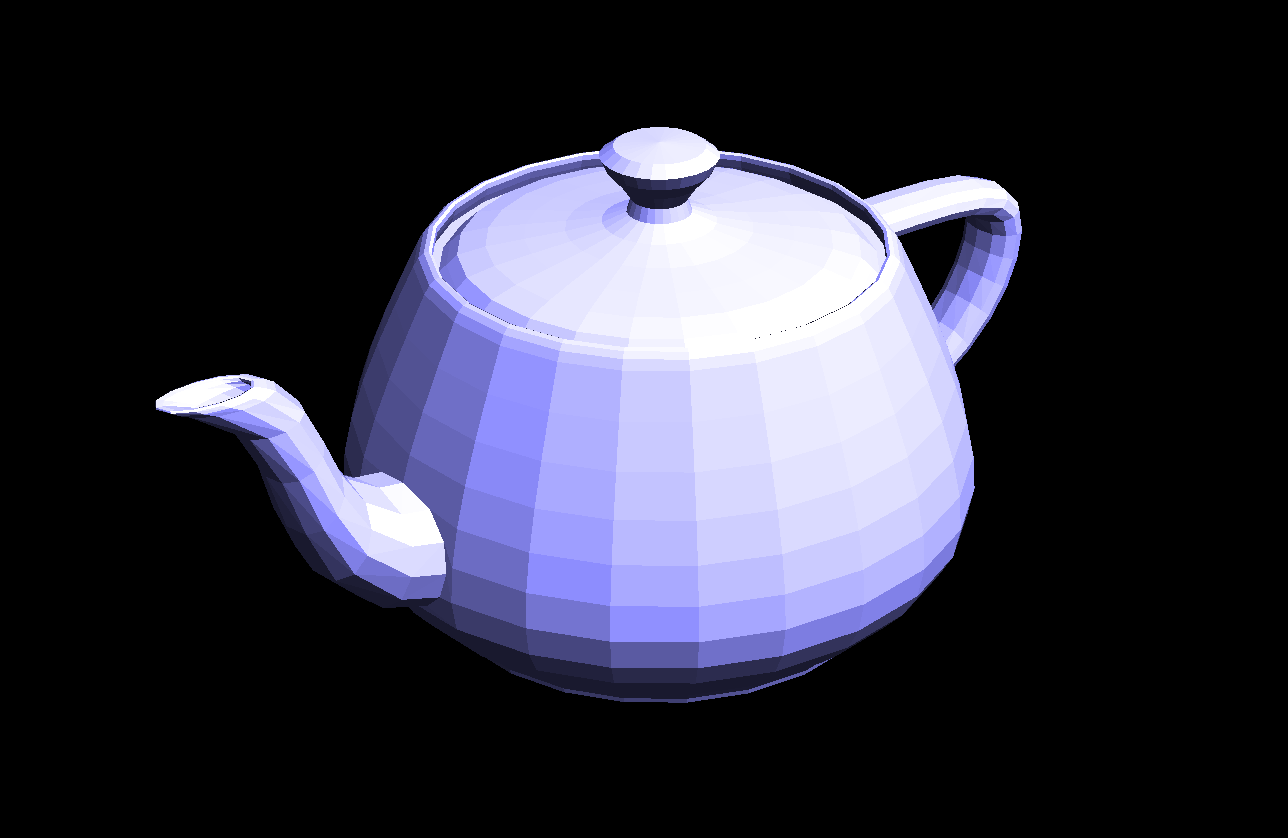This is with face normals while toggling the GLSL Shaders.This is with the average vertex normals while toggling the GLSL Shaders.

### Part 4: Half-edge flipPart 4 started the more difficult parts of the project. Flipping edges meant keeping track of a lot of pointers and ensuring the correct reassignment of each pointer that was changed. To approach this, I started by loading literally every variable for a particular pair of triangles into memory. For instance, I loaded all 9 halfedges, all 4 vertices, all 2 faces, and all 4 edges. Then, I drew out a diagram of where each new pointer should point after the flip. I used the CMU document for reference.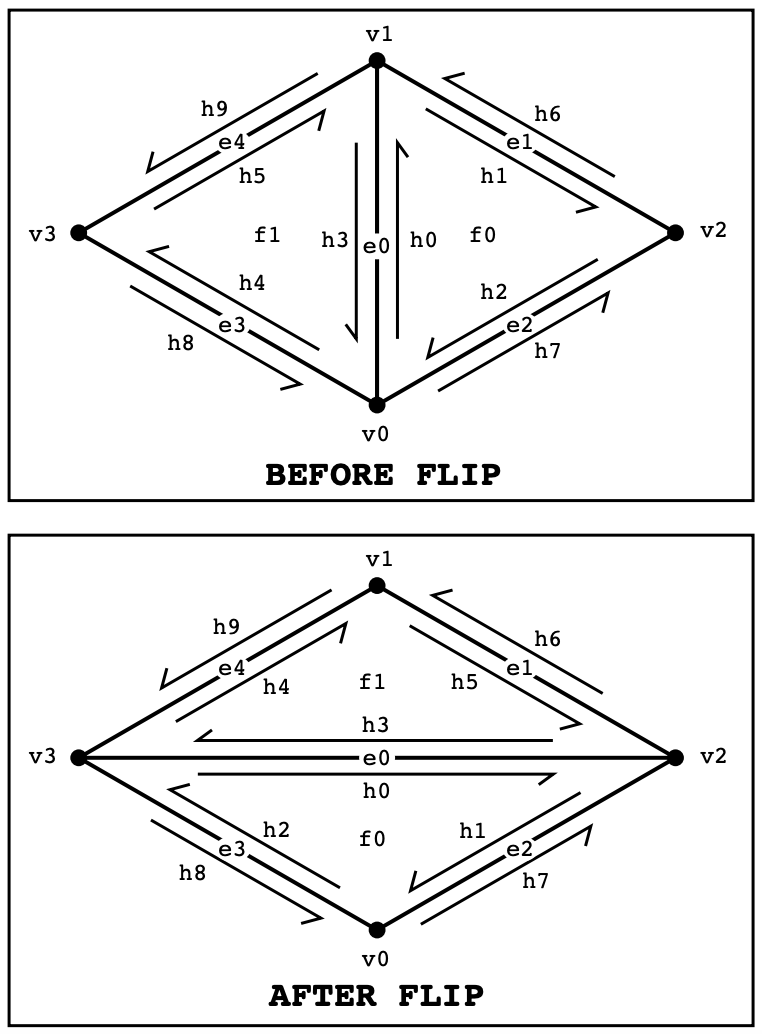Before using the setNeighbors function, I first reassigned each indivdual pointer for every object so that I could keep track of the number of reassignments and not worry about reassigning things like the faces for external half-edges that should not be changed anyways. Then, I went back and after confirming it worked, I used the setNeighbors function.The original edges.The flipped edges.

### Part 5: Half-edge split

My approach to solving half-edge split was almost identical to the process for flipping edges. The only caveat is that in splitting, we have to build one new vertex, two new faces, three new edges, and six new halfedges. So after loading all the variables, I create new variables for these new pointers, and then procede to perform the reassignments to all the variables I loaded in the memory. I also drew out the paths and reassignments of all the variables on a whiteboard before reassigning them.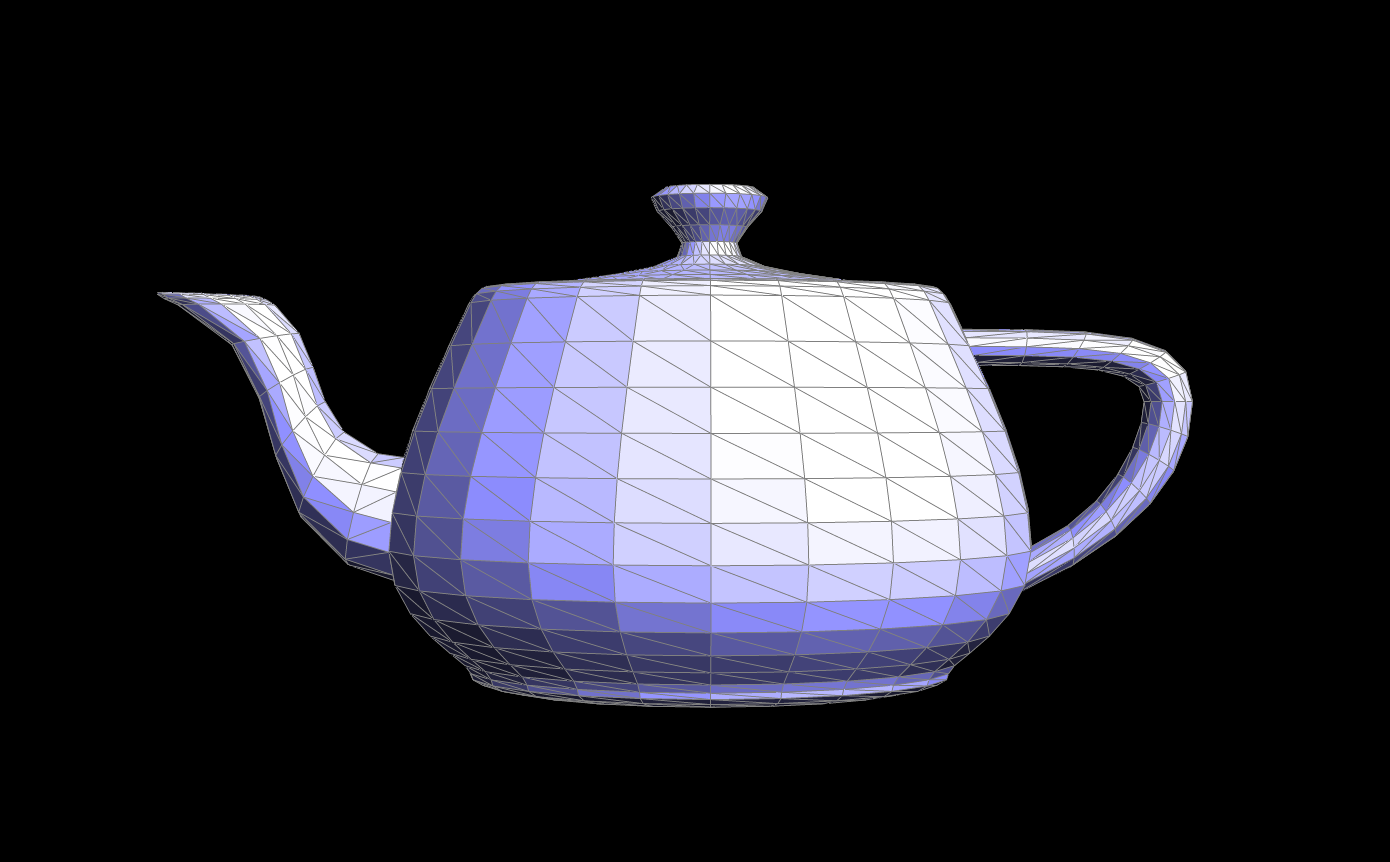The before picture of our teapot.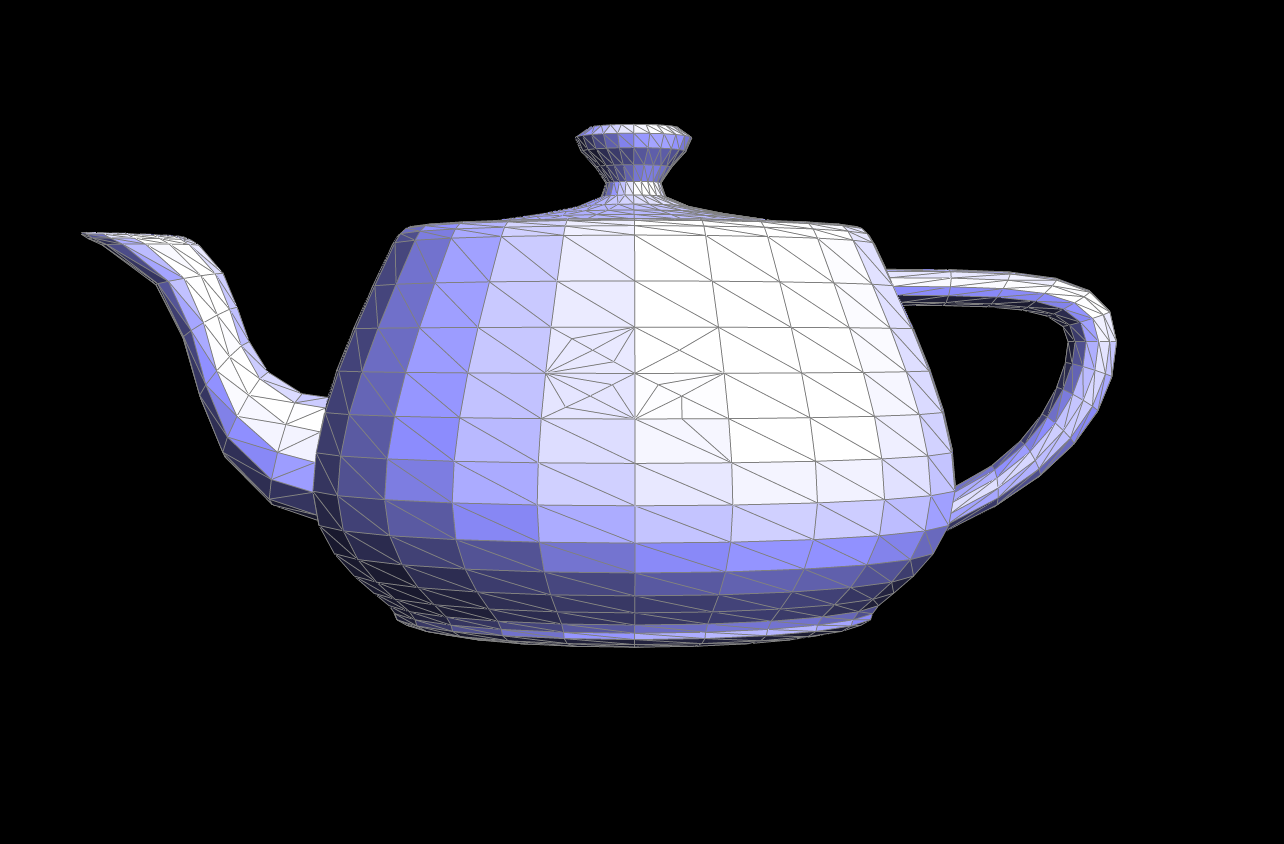Performing split operations on a bunch of edges in a variety of different orders and directions.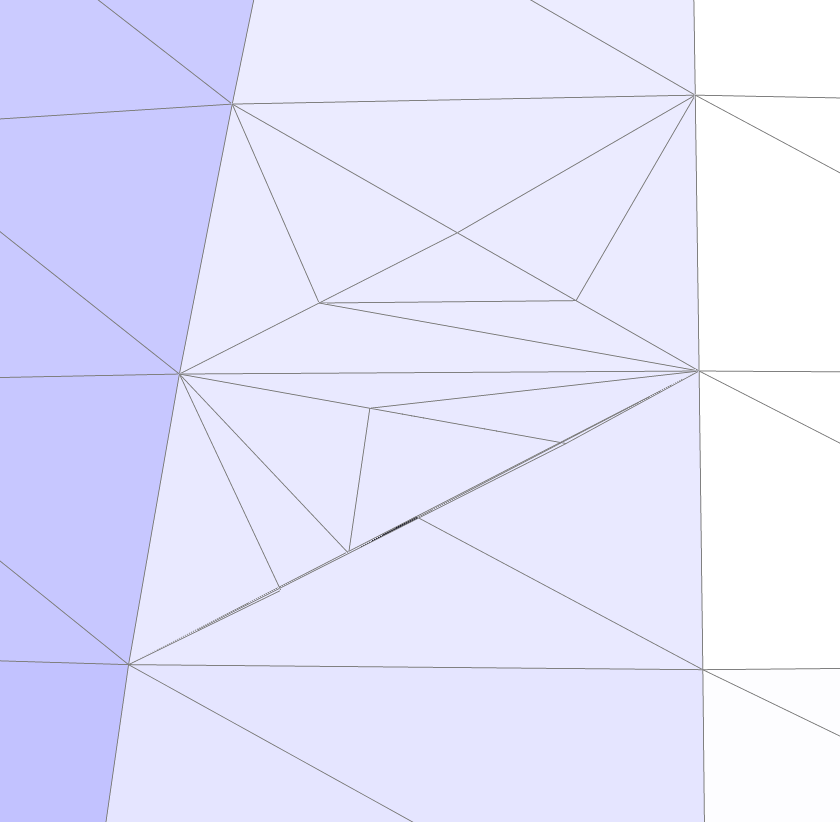A closeup of flips and splits and its result.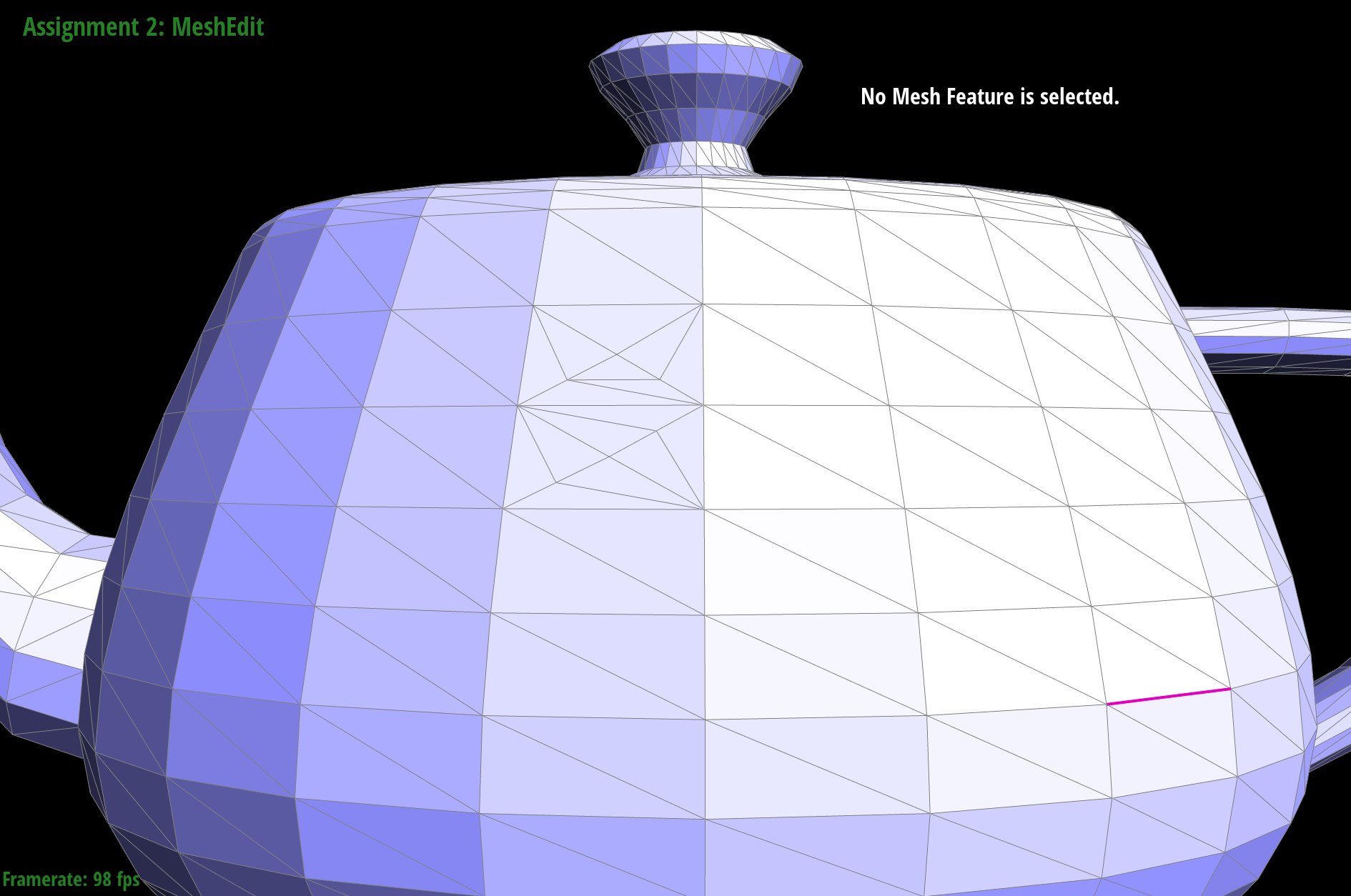A zoom out of flips and splits and its result.

### Part 6: Loop subdivision for mesh upsampling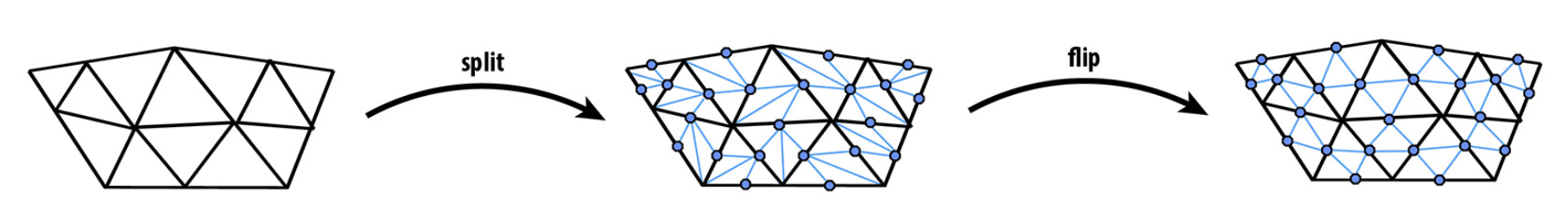Part 6 was probably the toughest of all the parts, and debugging was rather difficult as well because upsampling took advantage of the flips and split operators I wrote earlier for our meshes. Which meant that my errors could have been anywhere in the three largest functions I wrote. Much of the beginning was formulaic, following the weighted position of new vertices based on old vertices was as simple as finding the degree, calculating the neighbor_position_sum (a sum of the positions of all the neighboring vertices) and applying a formula:I did run into a bug where I was calculating the edge position wrong, but after re-reading the spec a good number of times, I found my mistake in the midpoint calculation and the images turned out ok. At the very bottom are some failures :P.
Another interesting issue I ran into was how to avoid using the isNew check in my while loop. First, I tackled this by simply counting the number of edges in the original mesh in an earlier loop, and only allowing my edge splitting loop to run over the pointers in the range of the original edges. However, after a trek to Office Hours, I worked out a solution to this, to avoid checking the !isNew on an edge, we instead check to see if an edge is connecting two old points. If it's connecting two old vertices, then it is an edge that still needs to be split. All other cases would not need to be split, so we adjust the condition to check for that.

#### The results for cube.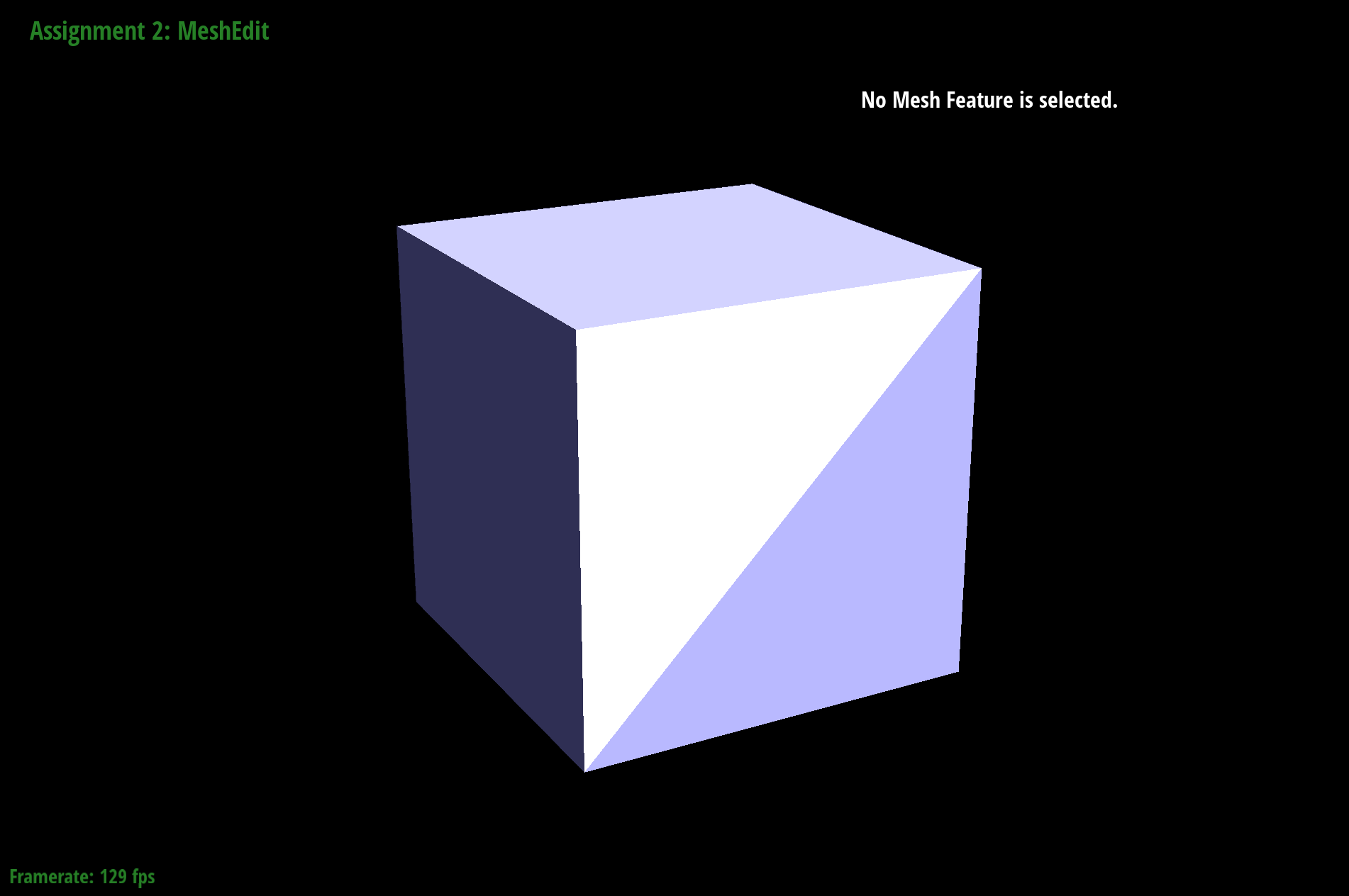The original cubeLevel 2 subsample.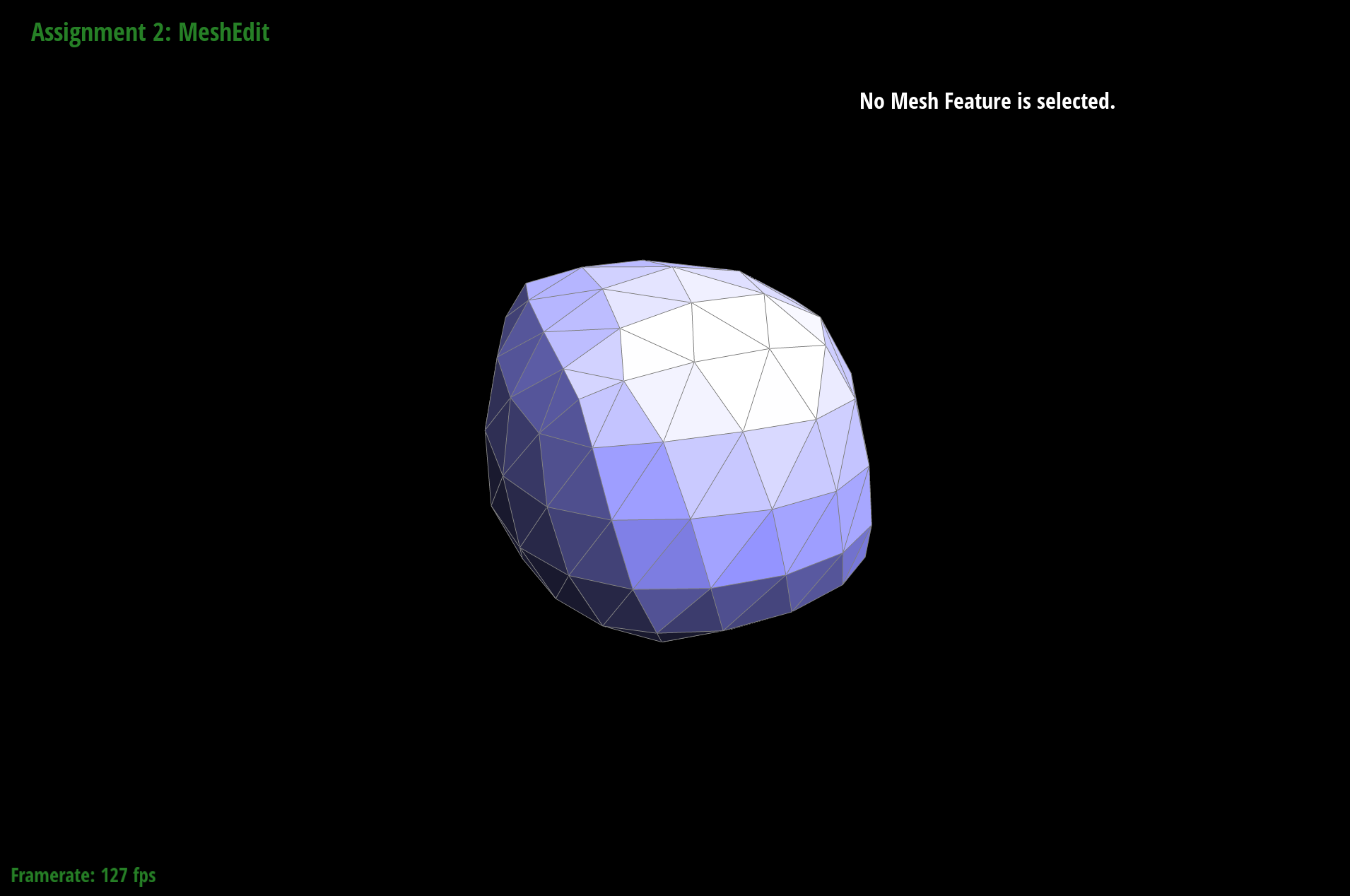Level 3 subsample.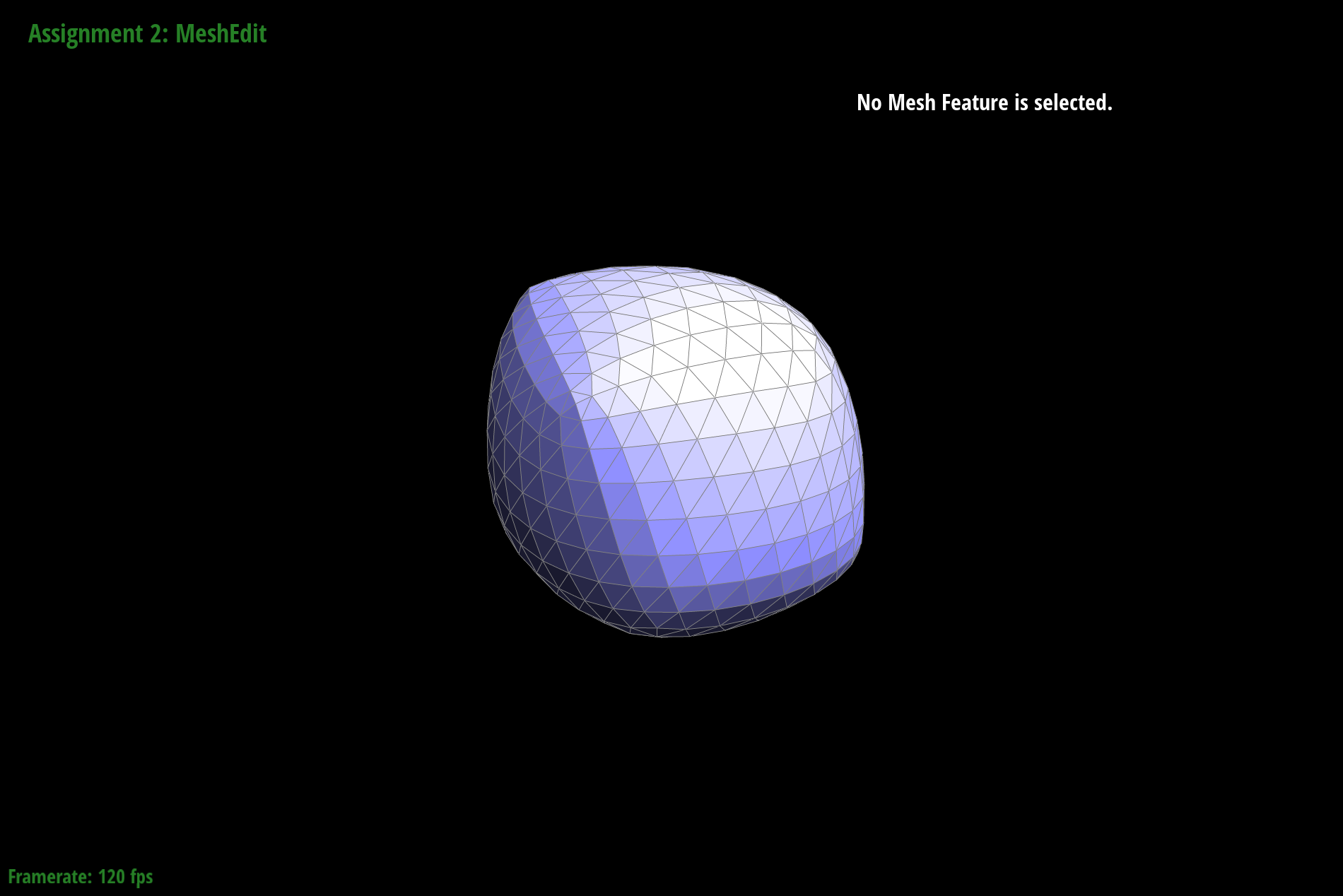Level 4 subsample.

#### The results for cube with the pre-splitting.

I pre-process by splitting all the edges that exist already so that I increase the resolution along the sharp edges, leaving more information before the subsample occurs. As we can see in the torus and isocahedron examples before, the sharp edges and faces end up shaping into curves due to the interpolation, but as is seen in the simple example of the cube, adding more information along the edges shows an improvement.The original cube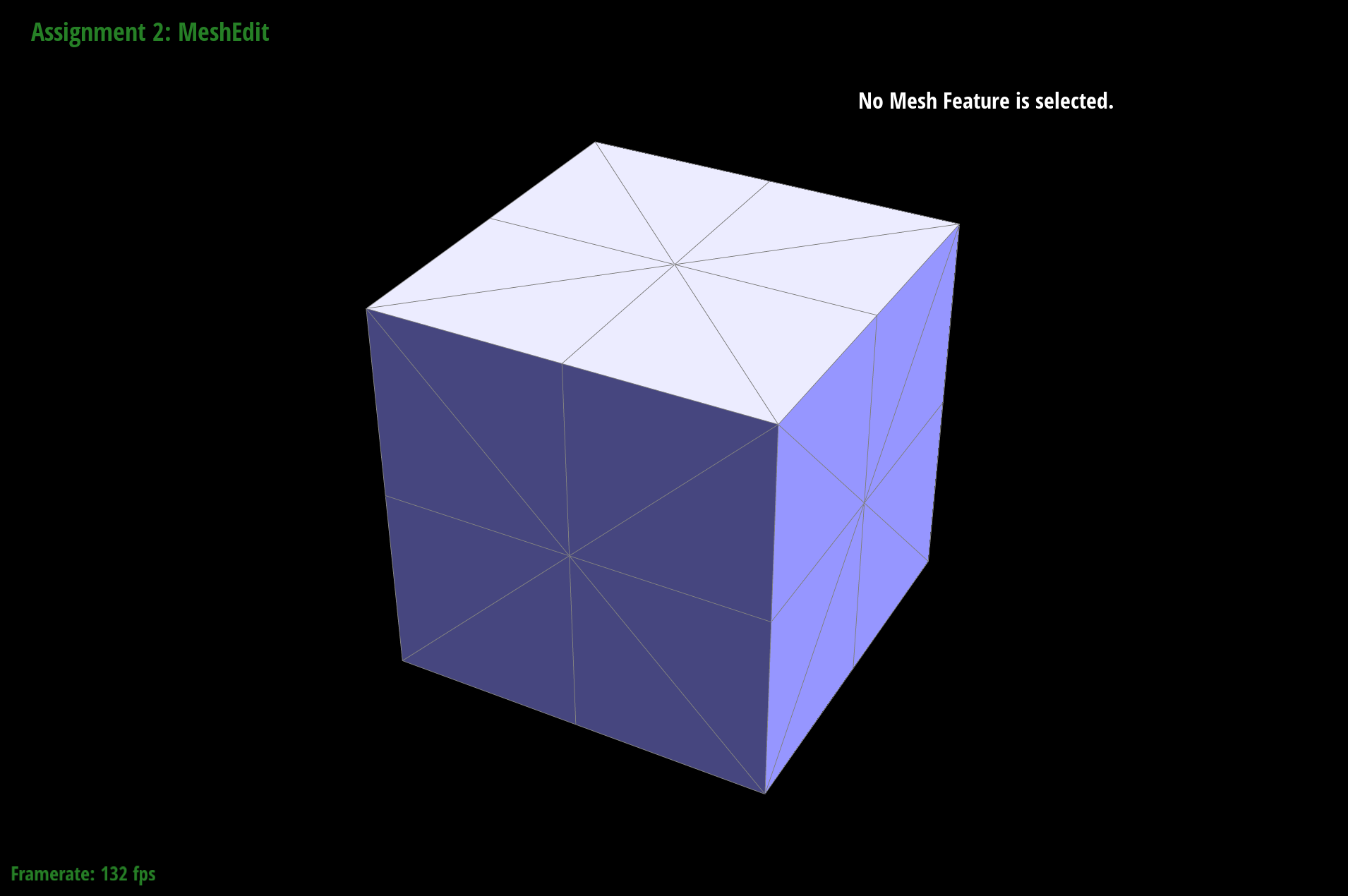My pre-processing technique to make sure the result is symmetrical.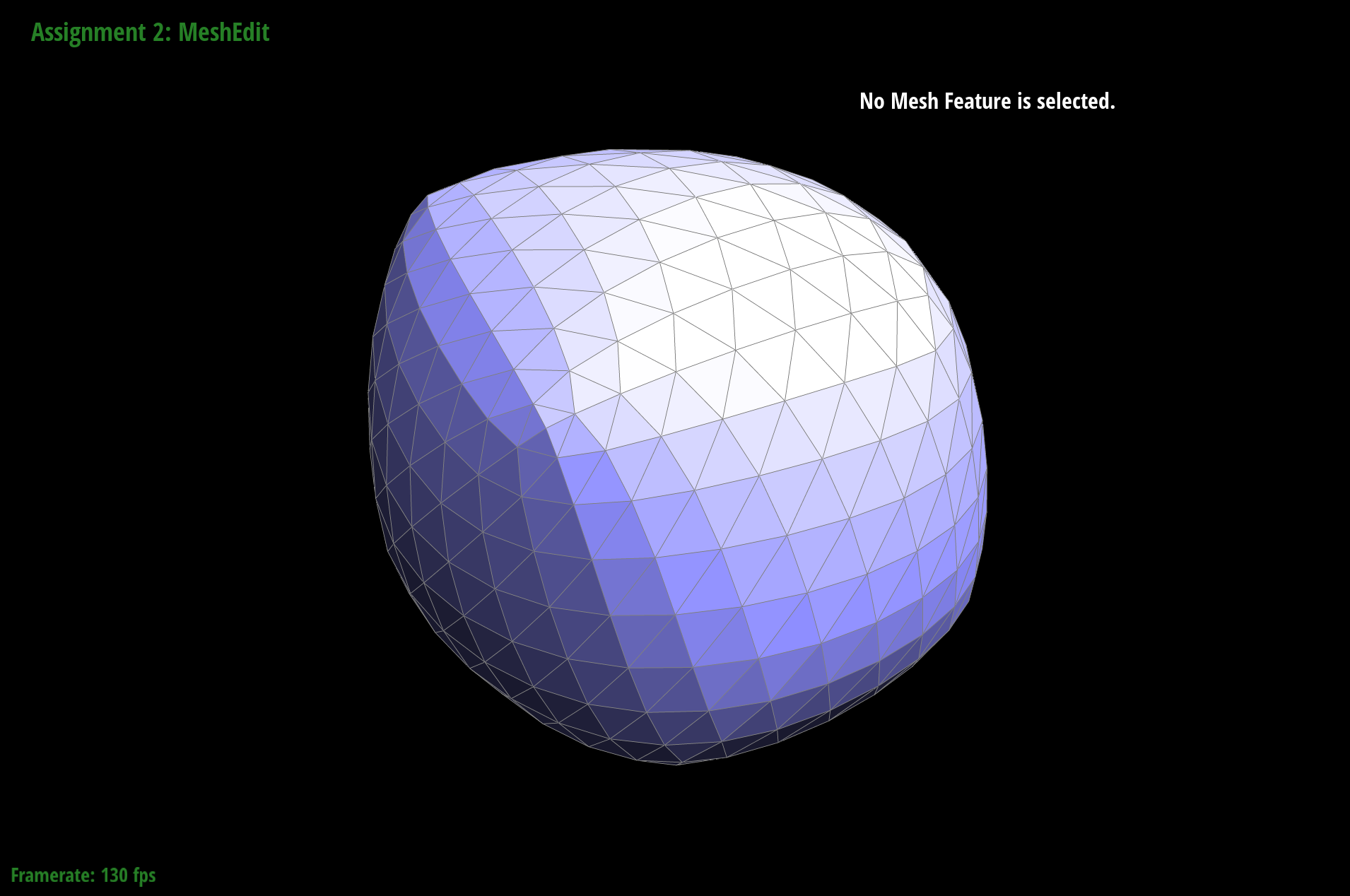When using the original cube, the asymmetric result.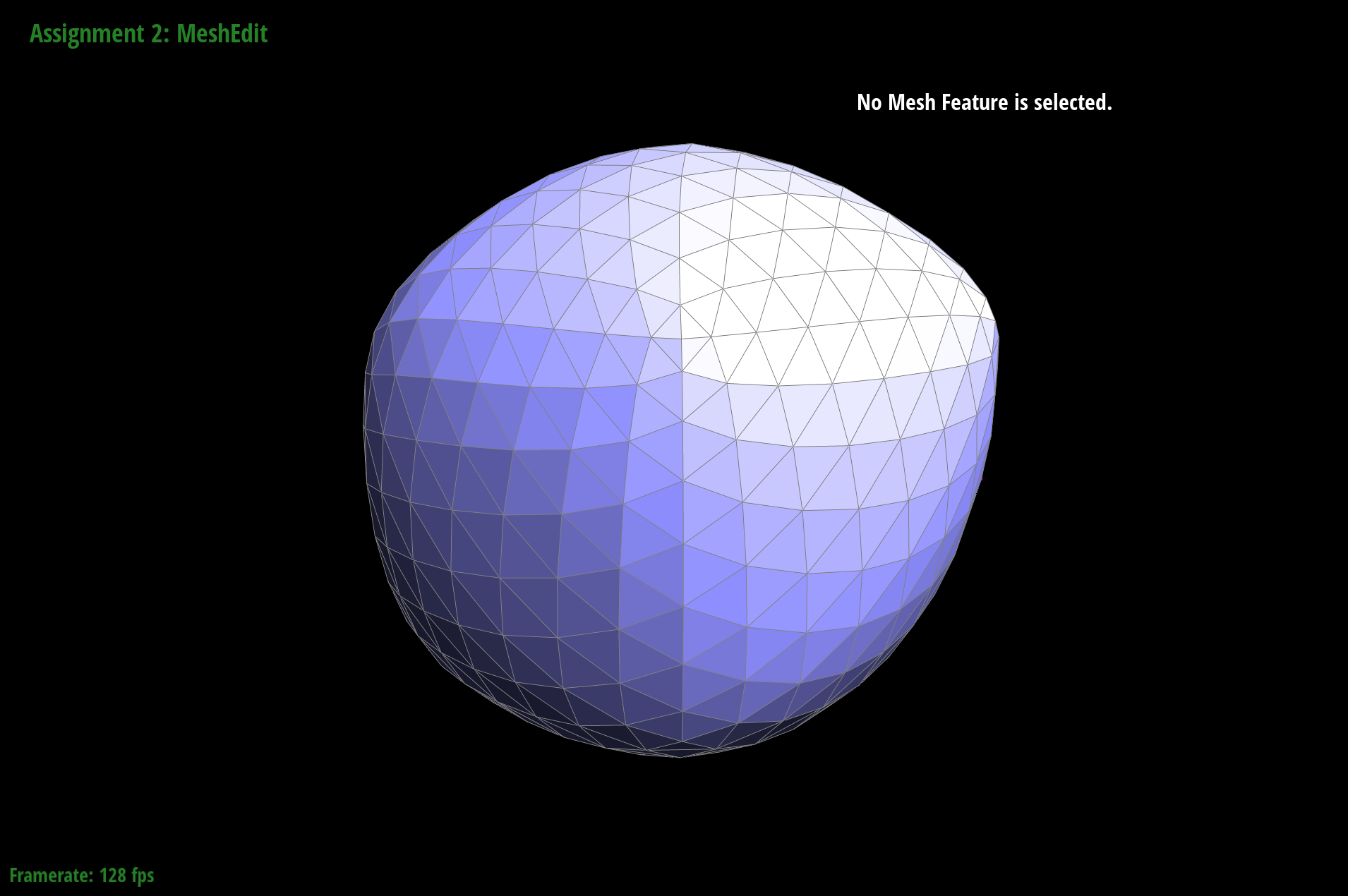Another perspective of the asymmetric result.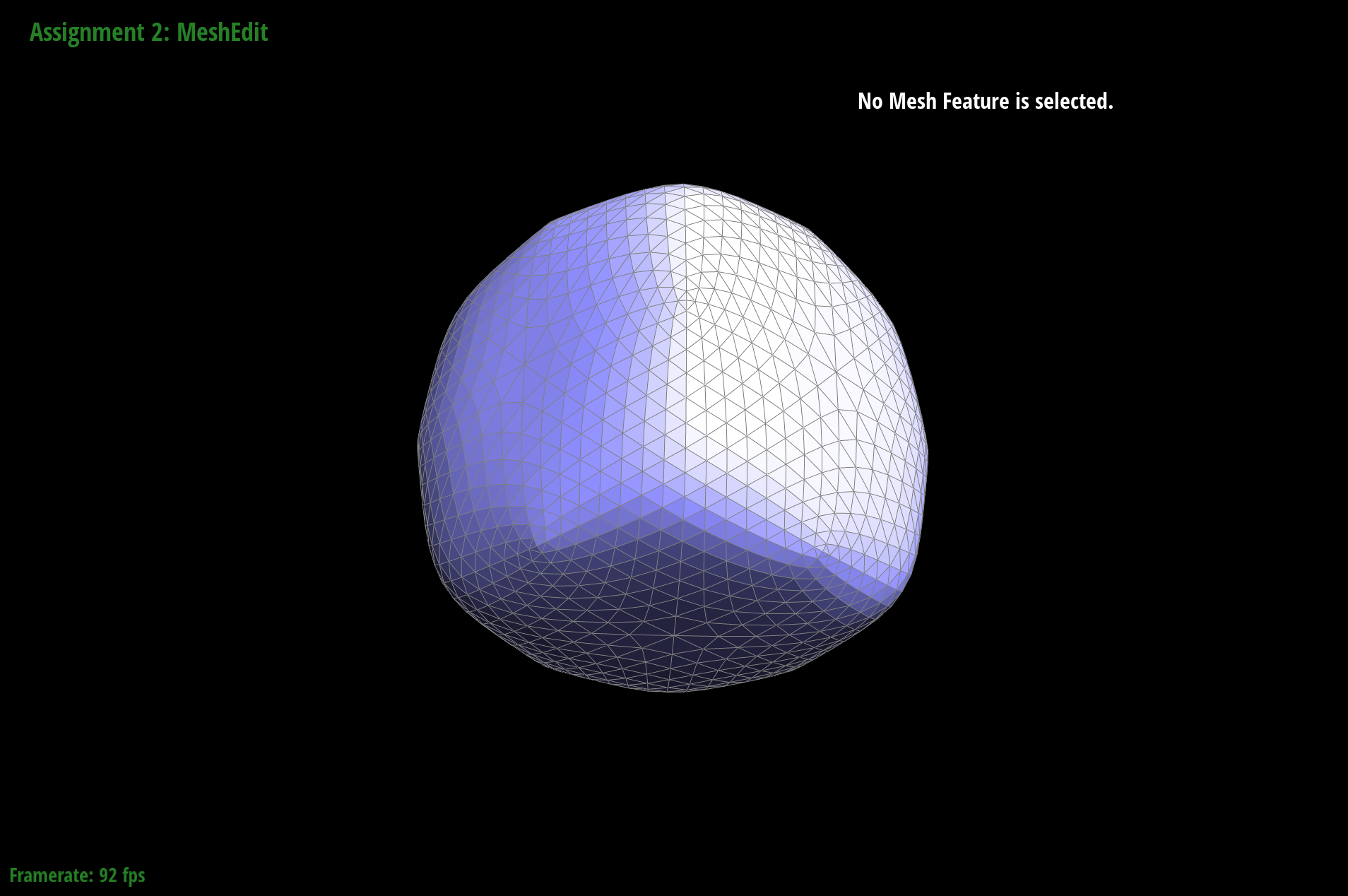When using the pre-processed cube, the symmetric result.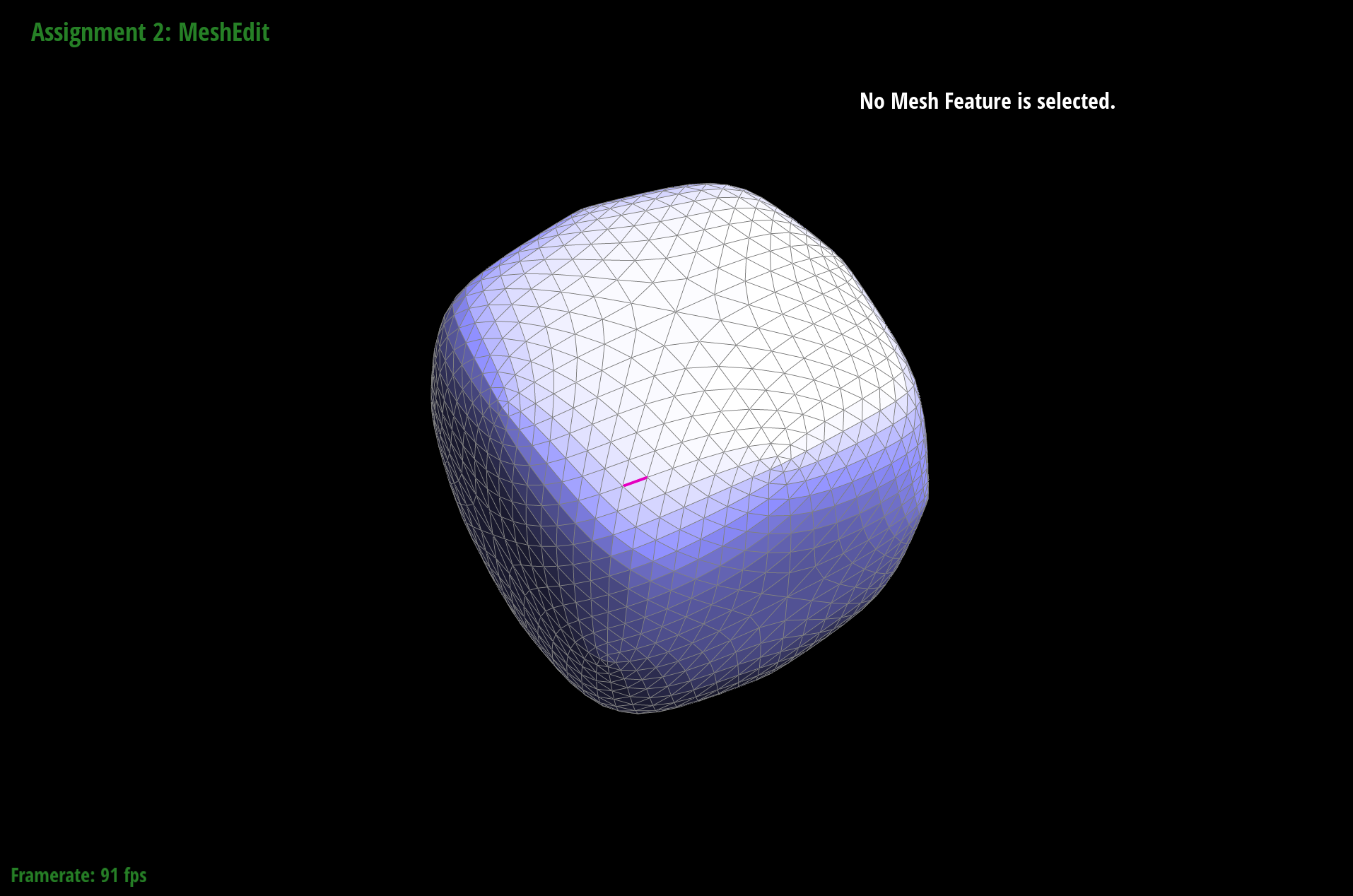Another perspective of the symmetric result.

#### Some other results of my subsampling

The Isocahedron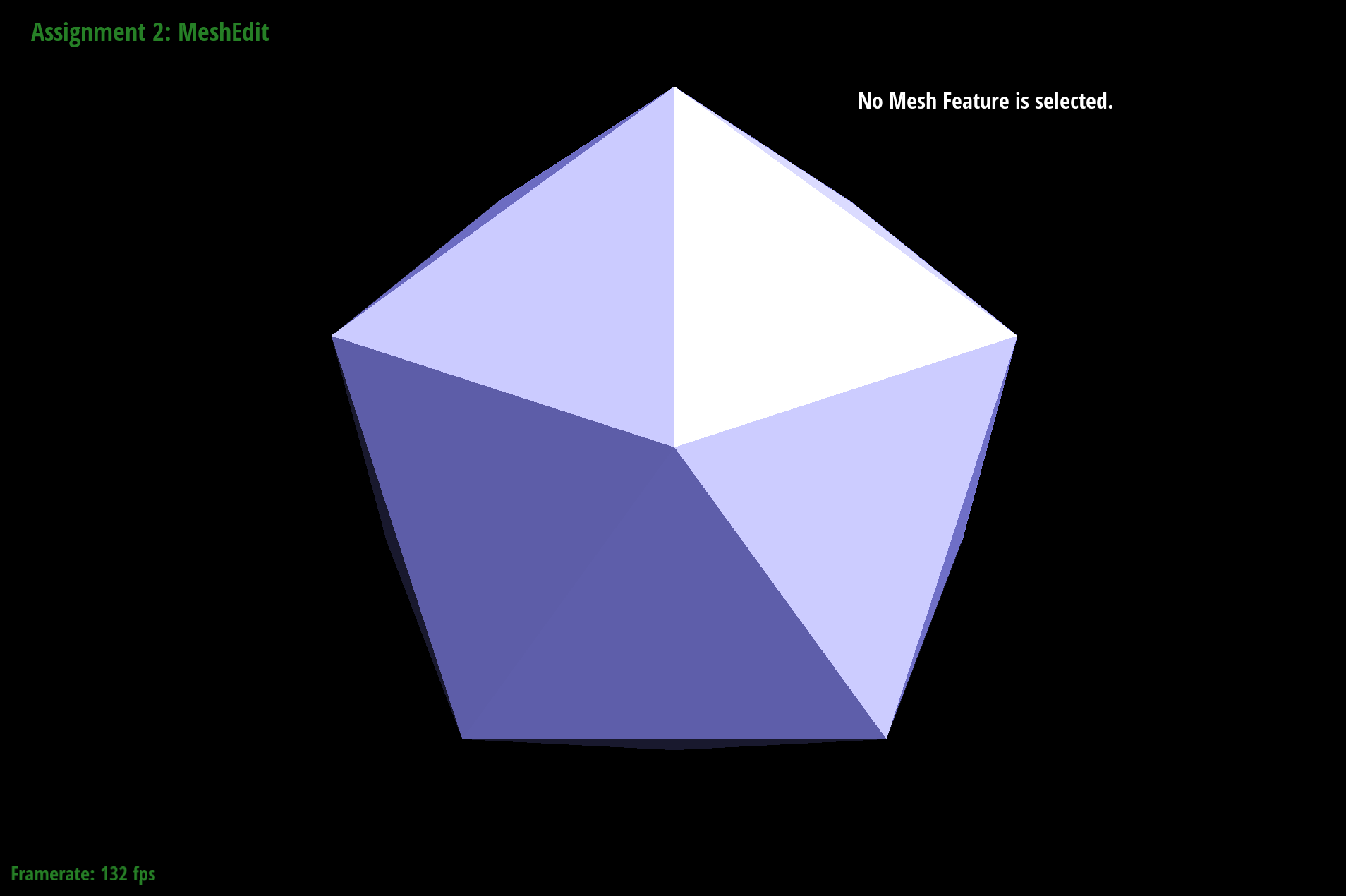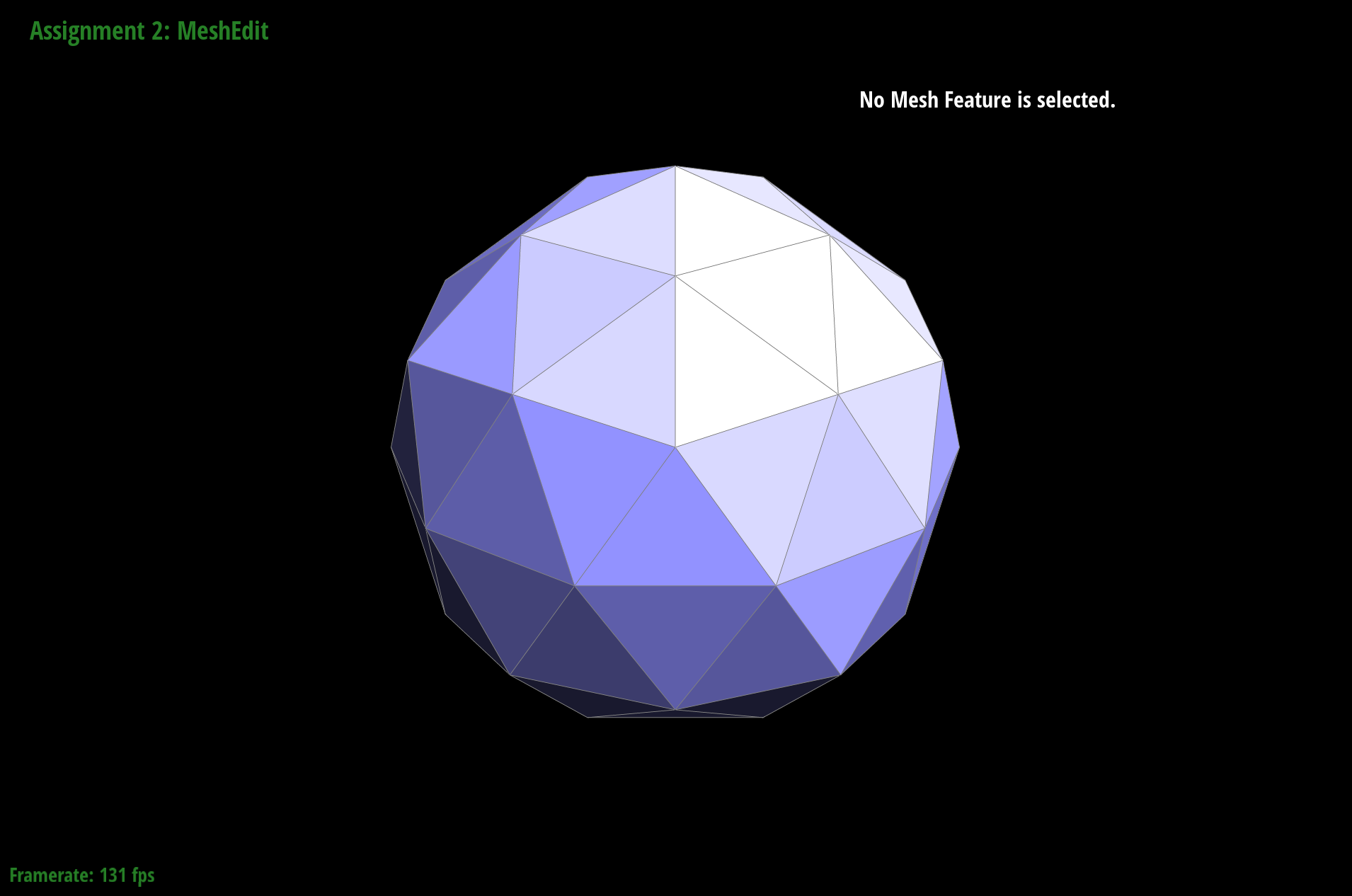The Torus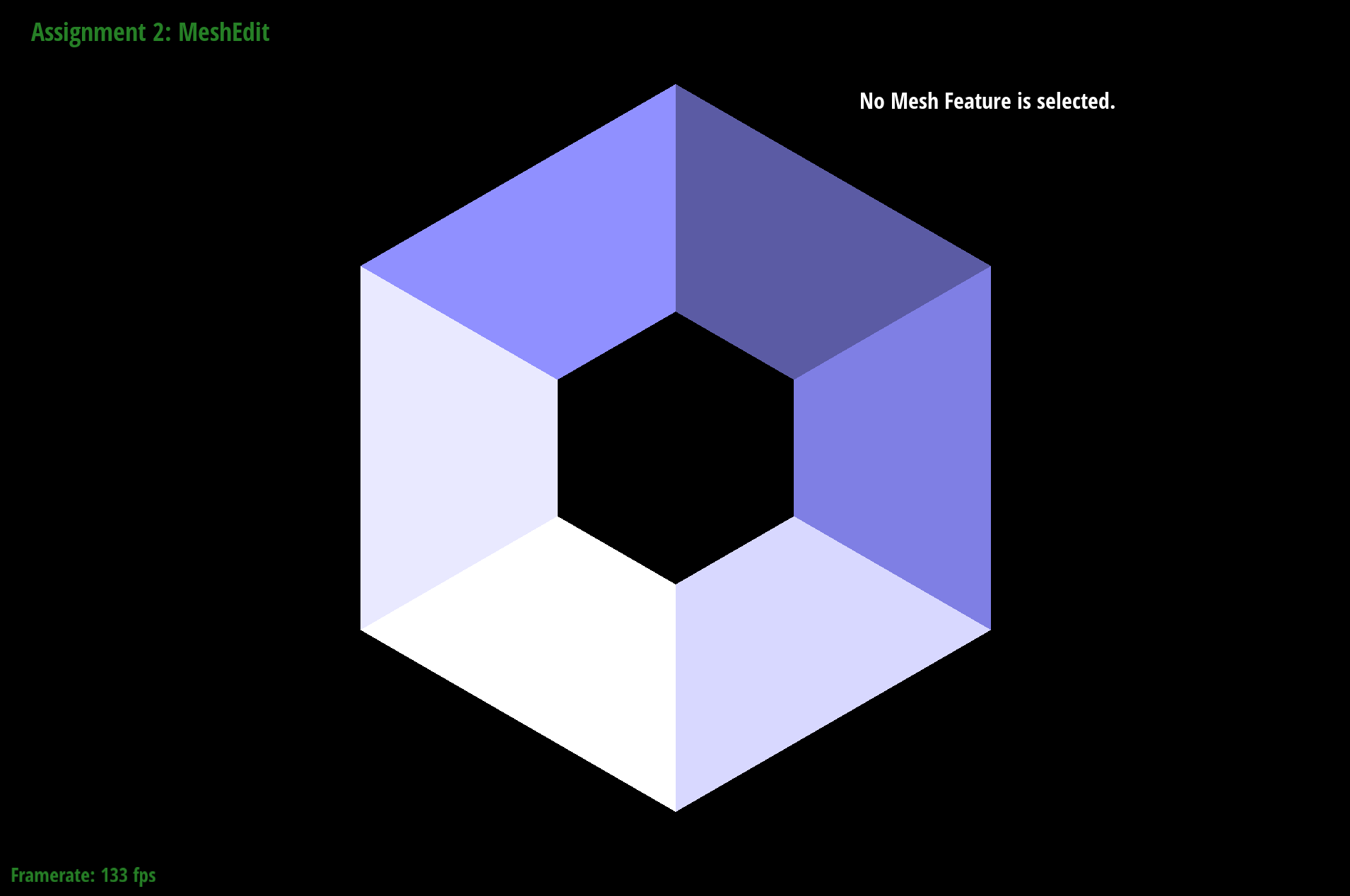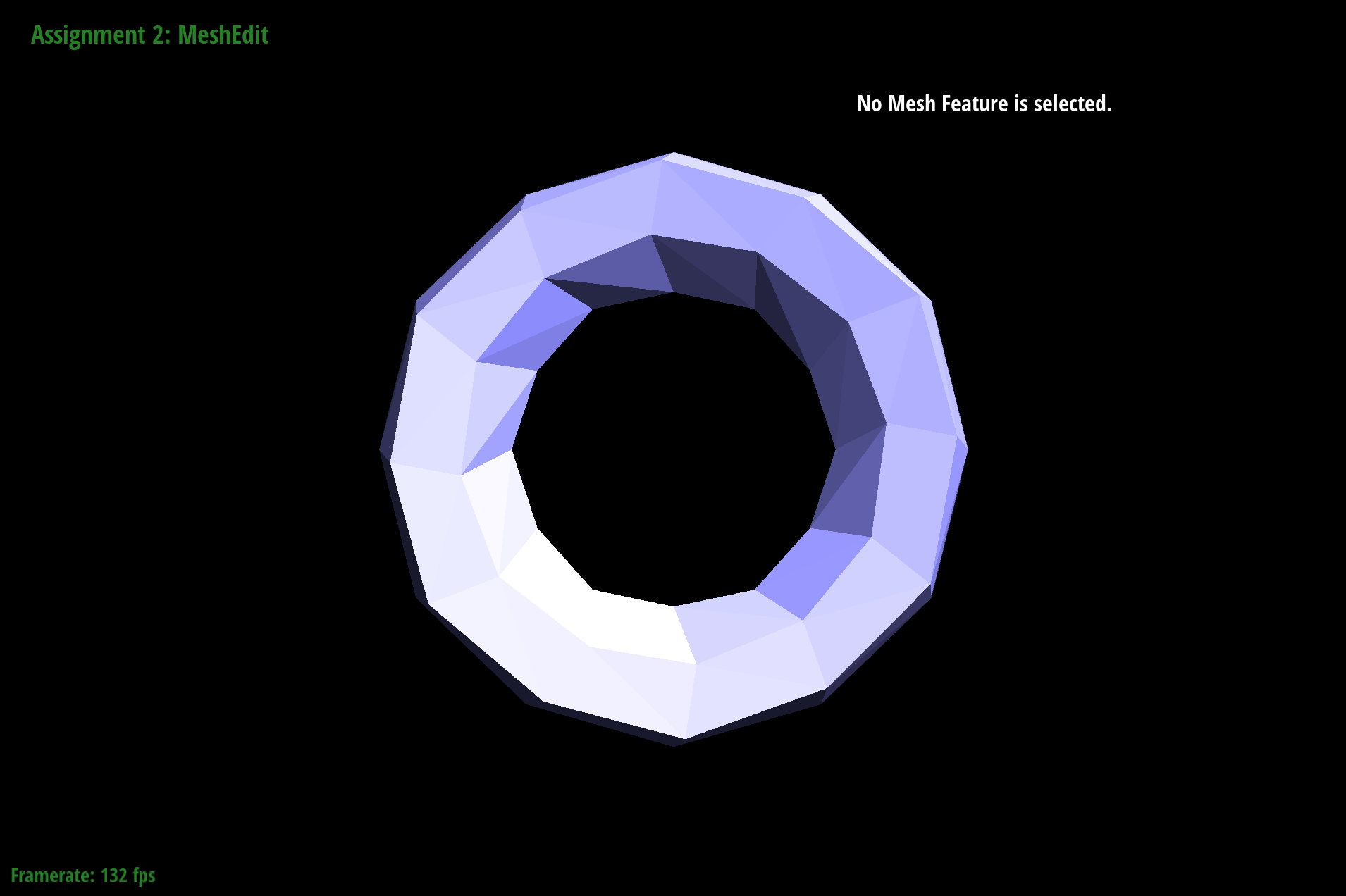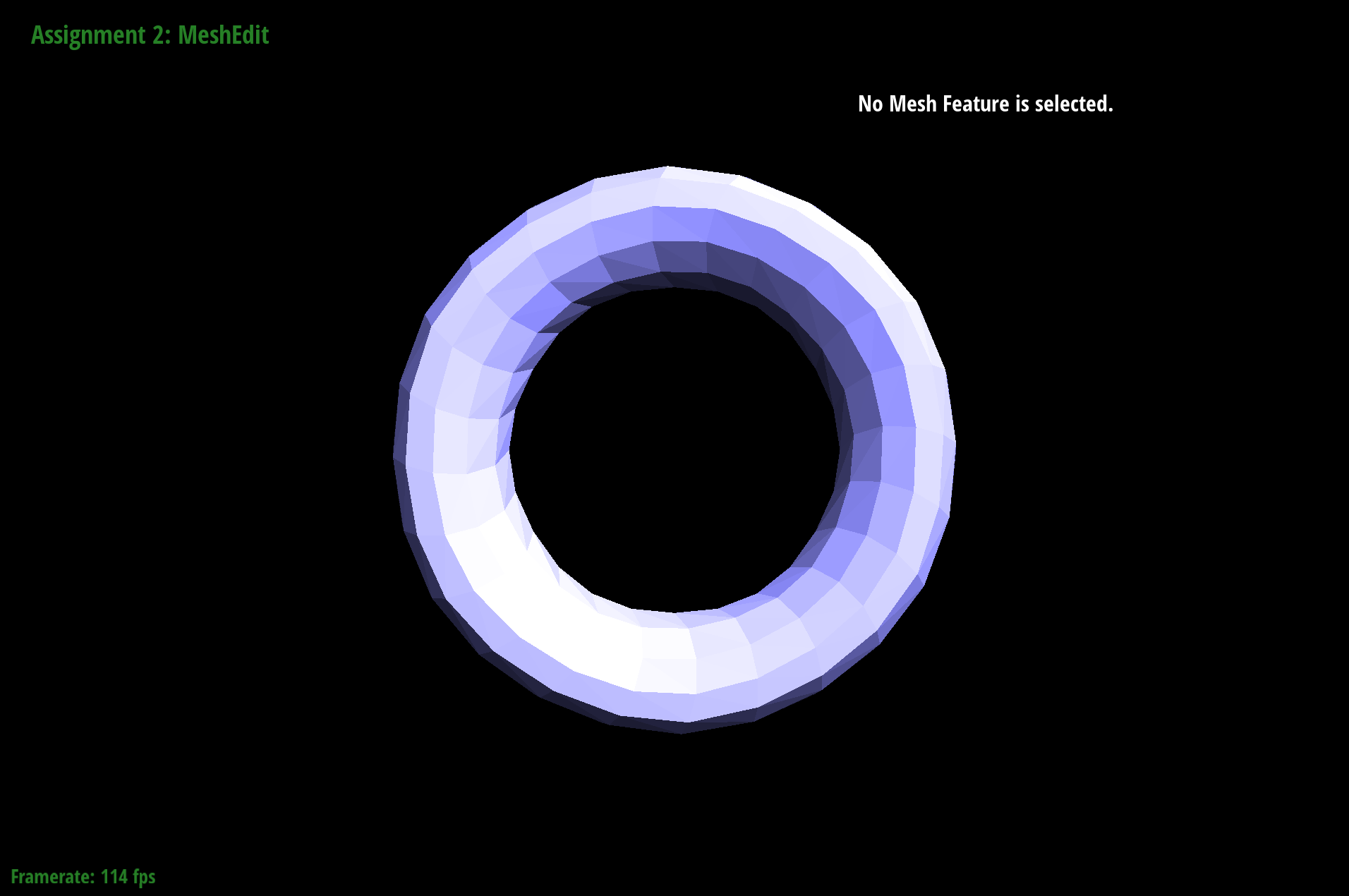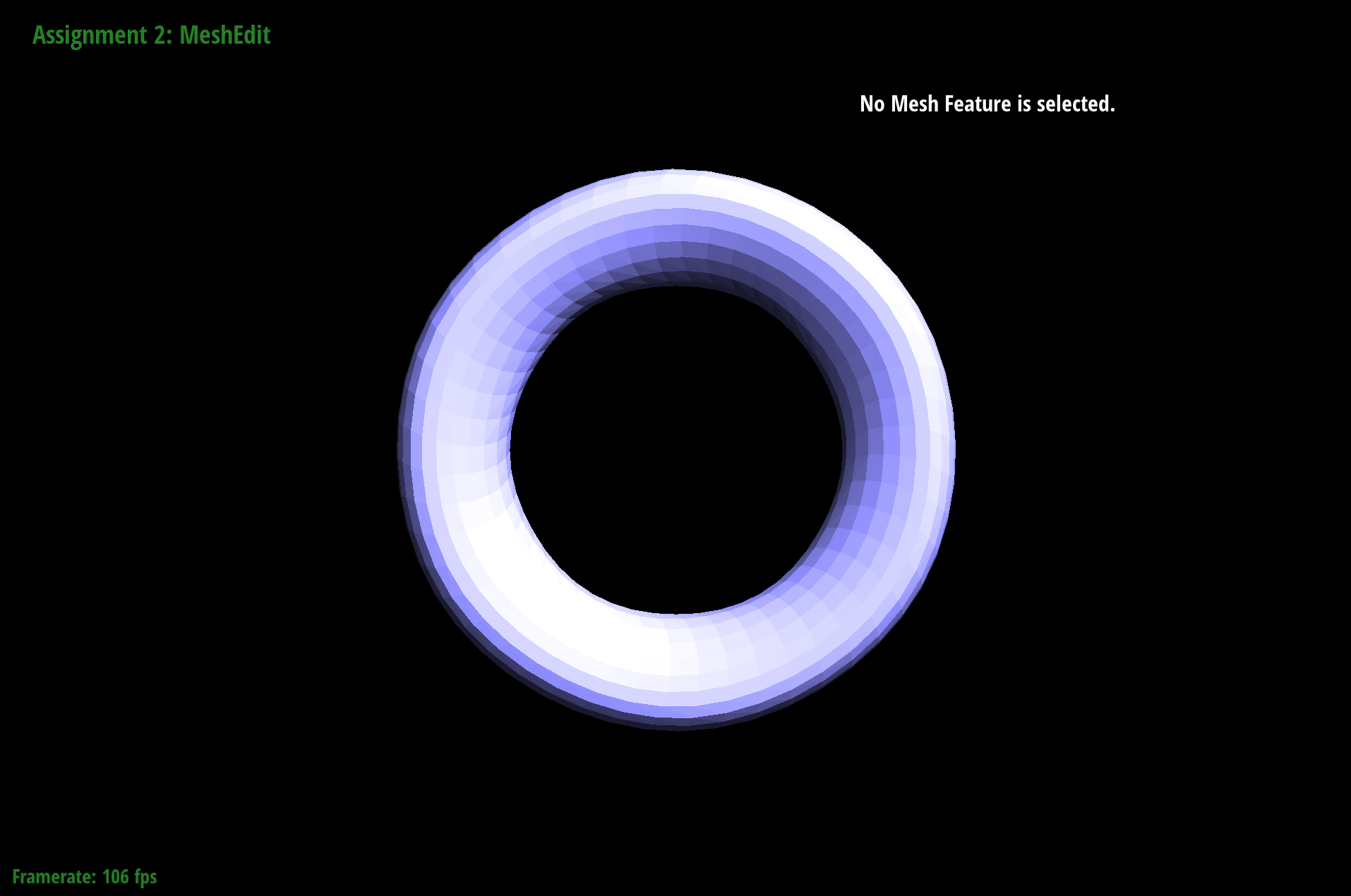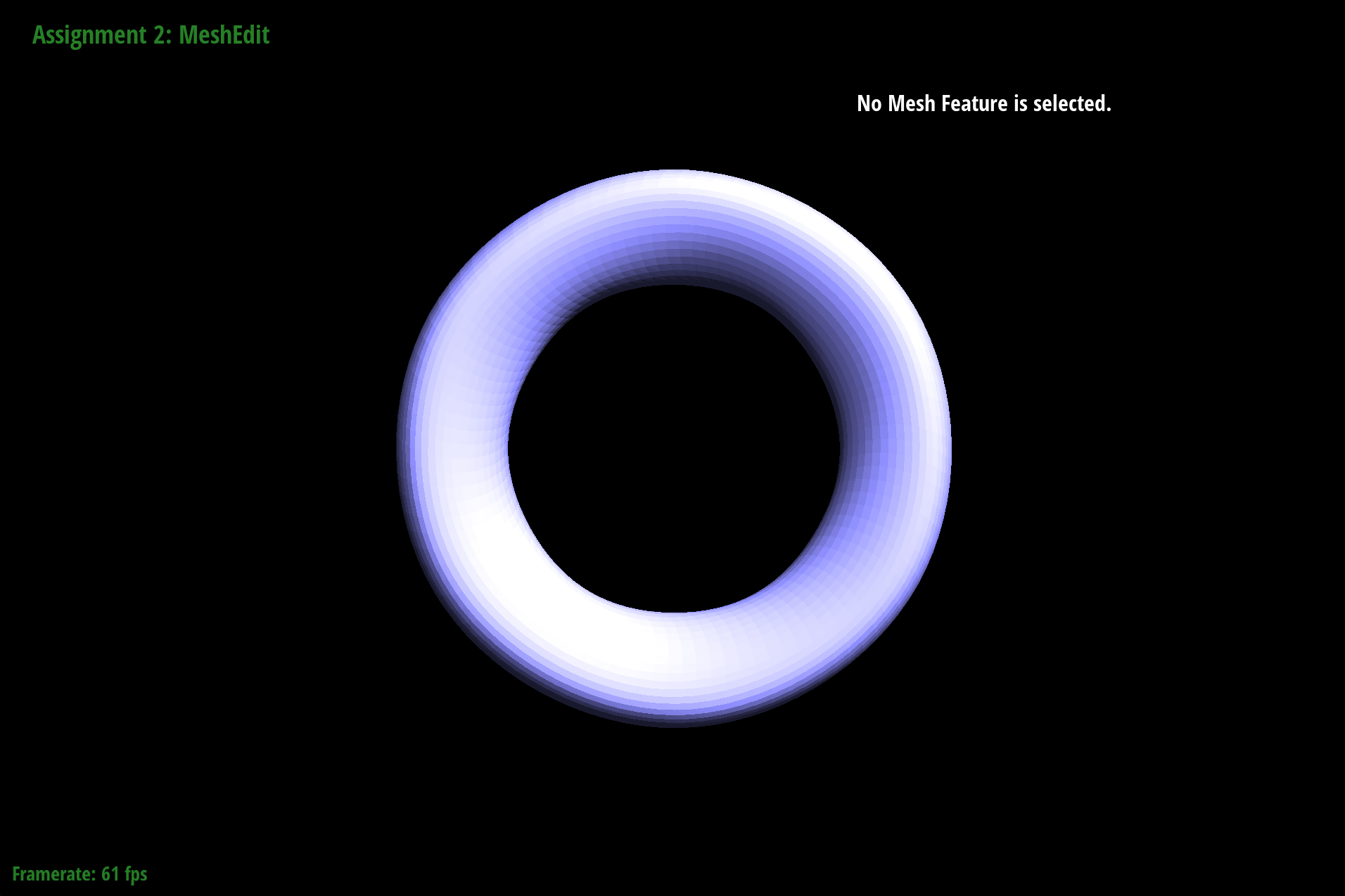#### Failures